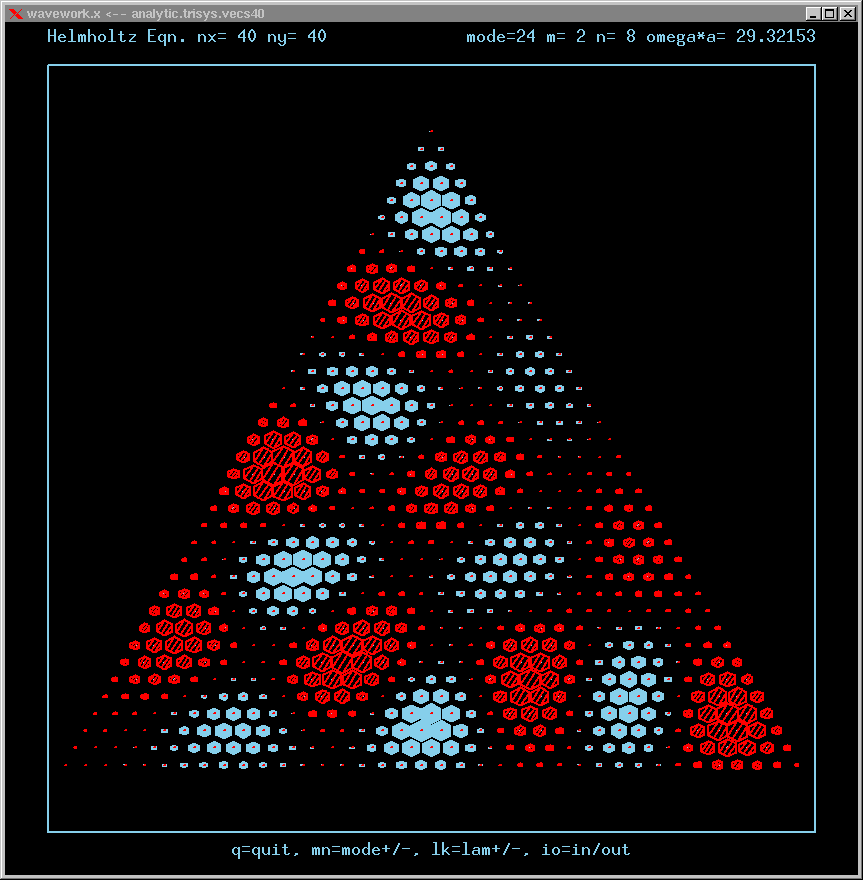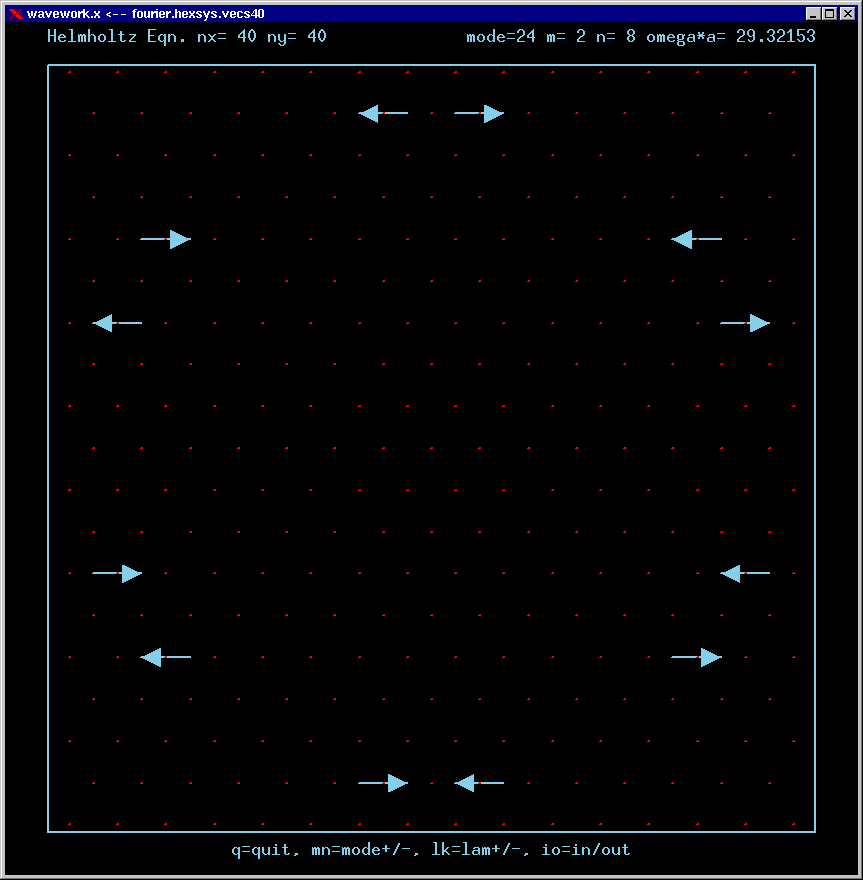## Triangular cavity modes 1 -- 24

Triangular cavity of edge length = a.
Filled with a refractive material of index = n.
Surrounded by a refractive material of smaller index = n'.
Boundary condition of zero field at cavity edge.

These are the real-valued wavefunctions and complex-valued Fourier Transforms of the lowest 24 modes, obtained from the known analytic solutions. They are displayed on a grid for convenience; the wavefunction magnitude at each position is proportional to the symbol size there. Red is positive, blue is negative wavefunction value.

Modes are labelled by indexes m,n.
When m is zero, the mode is single or nondegenerate.
When m is non-zero, the mode is doubly degenerate (has the same frequency as another independent mode of vibration).

The dimensionless frequency numbers on the plots are actually omega*a*n/c, where n is the index of refraction inside the cavity, and c is the speed of light in vacuum.

For the Fourier Transforms, the arrows represent the magnitude and phase of the Fourier amplitude at a chosen wavevector. The spacing of the grid points in Fourier space is 4*pi/3a. The entire Brillouin zone is hexagonally shaped, as shown in the FT of the first mode. The wavevector (kx,ky)=(0,0) is the point at the center of the diagram. Only the important inner part of the BZ is shown for the other modes.

A mode can be confined by total internal reflection as long as there is no FT amplitude propagating normal to any boundary. For example, using the lower boundary, a mode cannot be confined if there is FT amplitude along the negative vertical axis of the FT diagram. Applying this type of analysis, only the modes with m=0 can never be confined by TIR. Conversely, all modes with nonzero m can be confined, at large enough refractive index ratio (n/n').

Click image for a postscript file of that figure.

Mode 1 (ground state, nondegenerate) and whole FT Brillouin zone: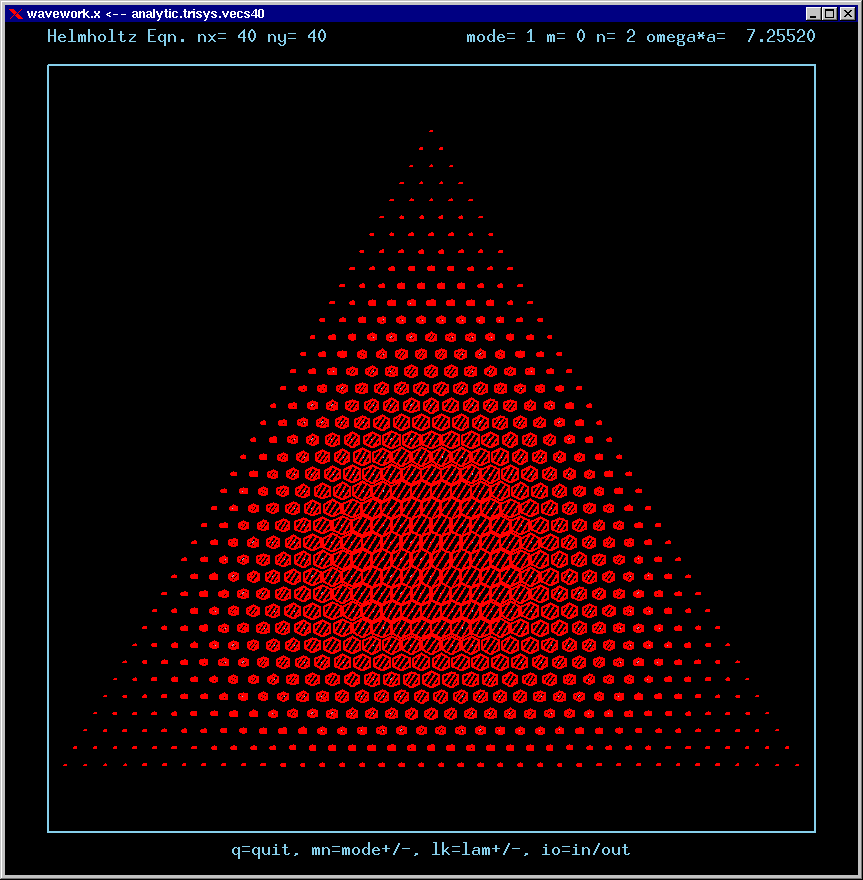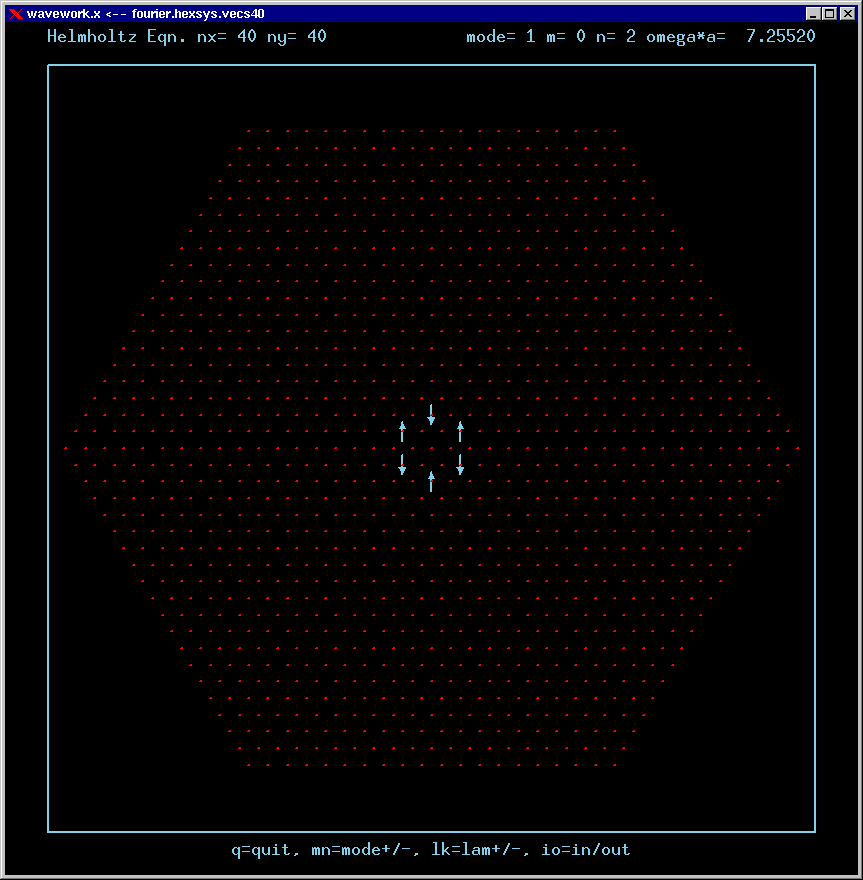Mode 1 (ground state, nondegenerate) wavefunction and zoomed Fourier transform: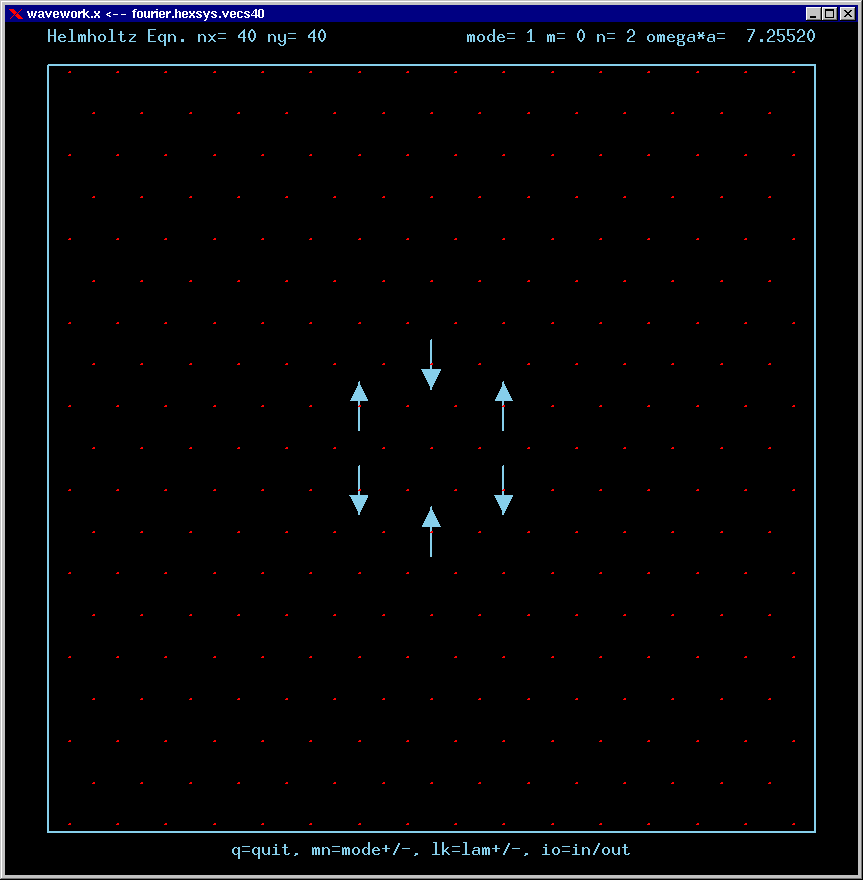Mode 2 (degenerate with 3) and its FT: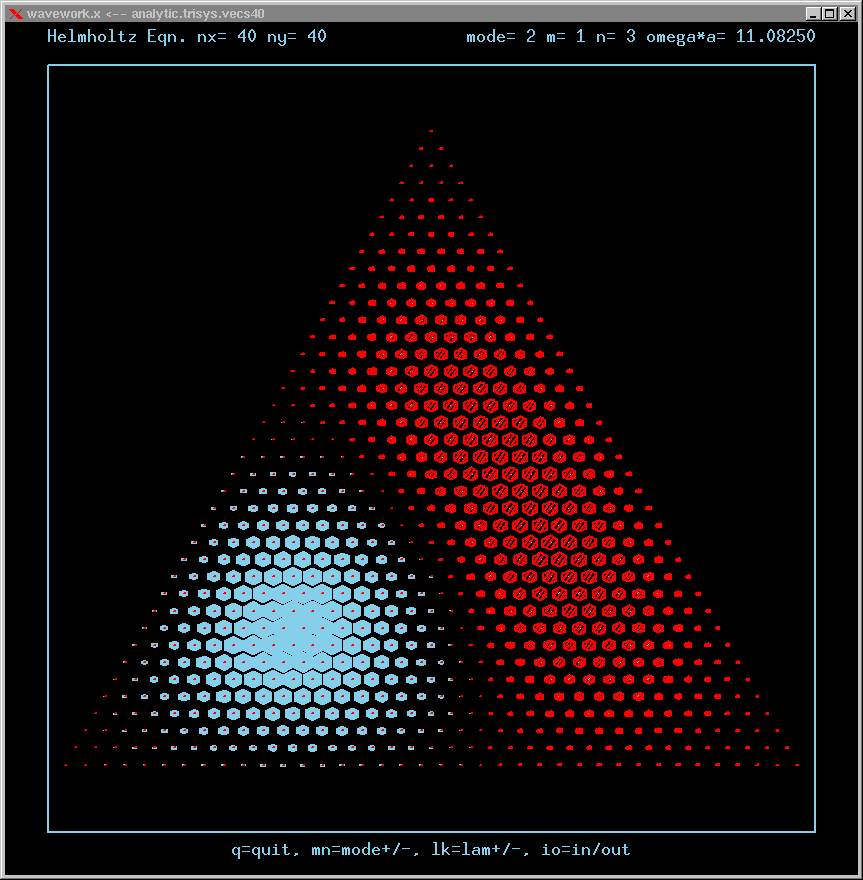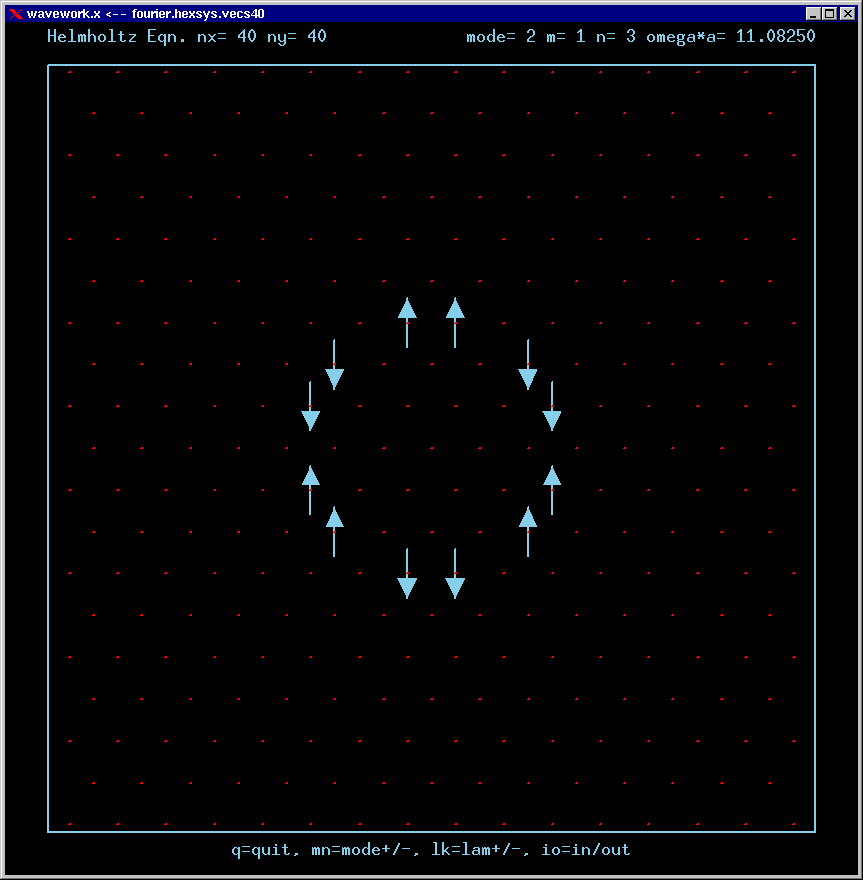Mode 3 (degenerate with 2) and its FT: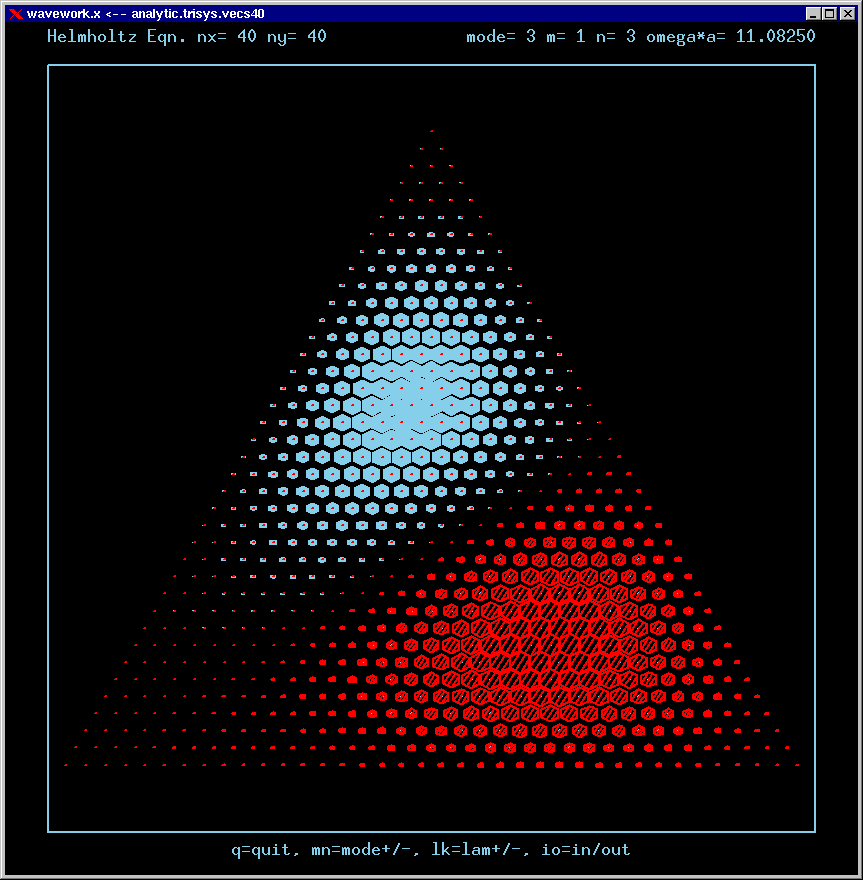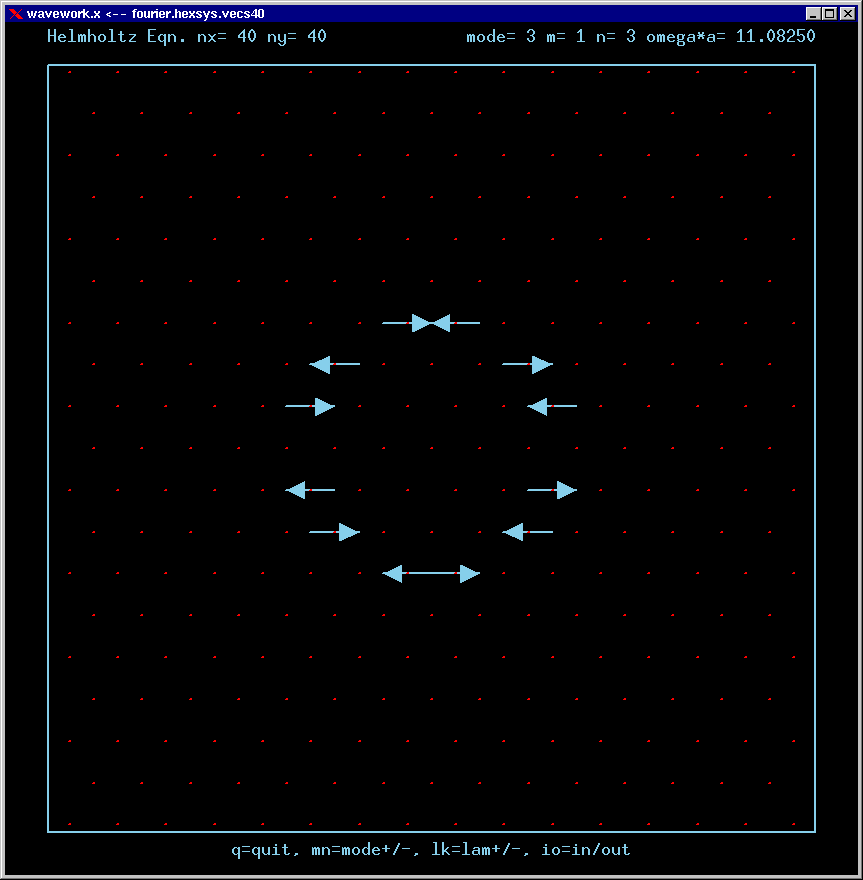Mode 4 (nondegenerate) and its FT: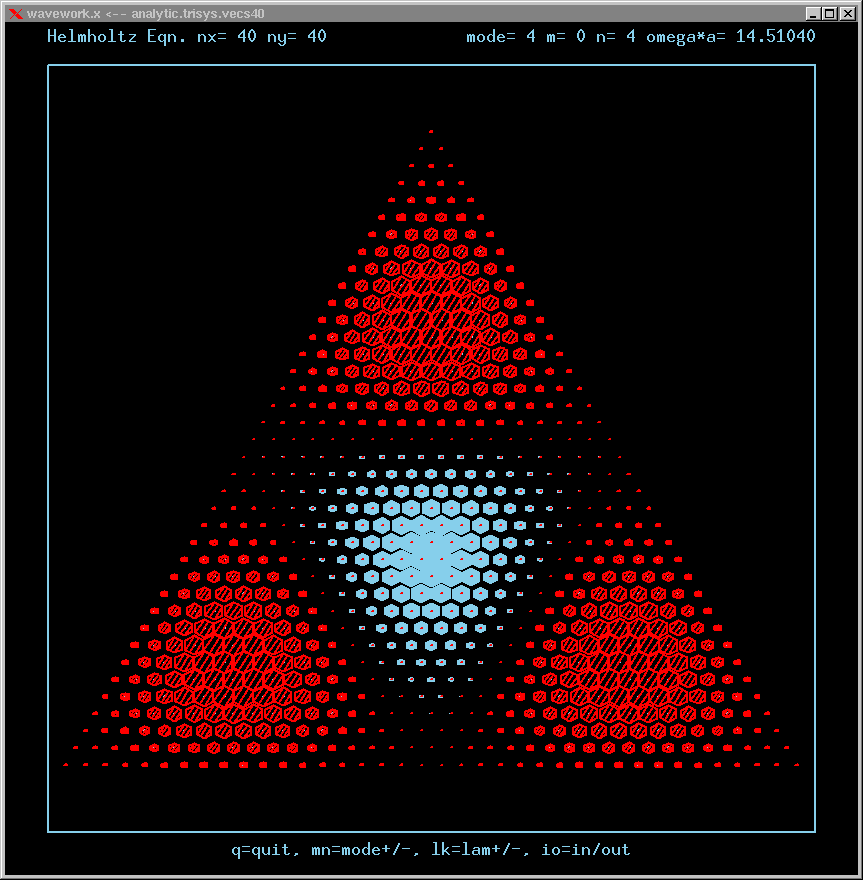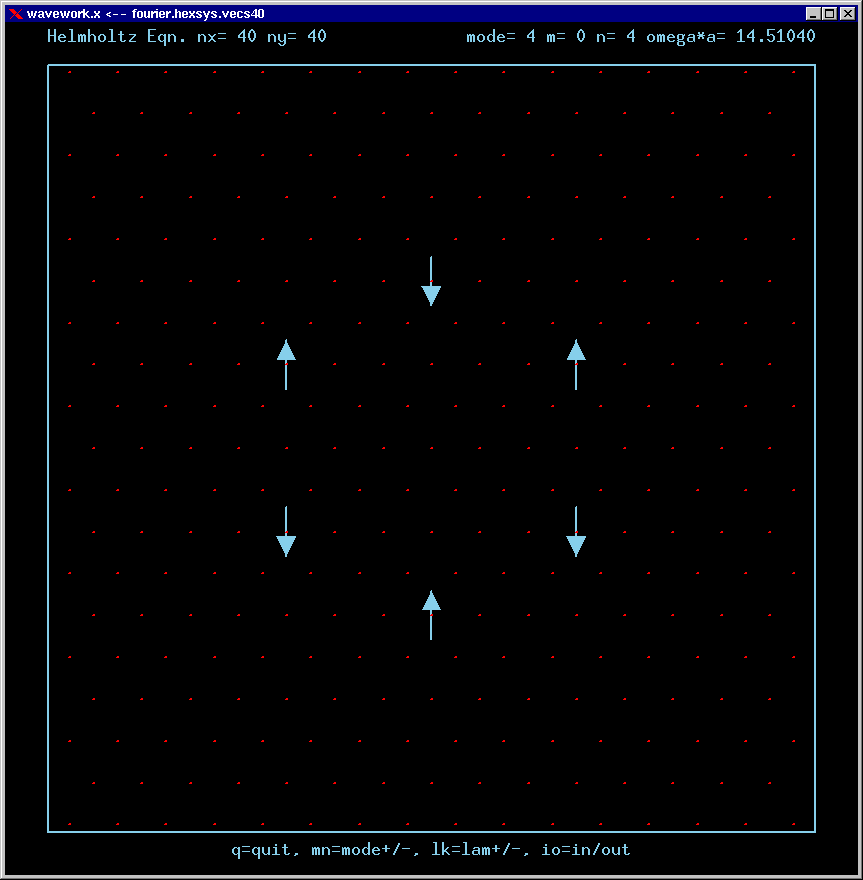Mode 5 (degenerate with 6) and its FT: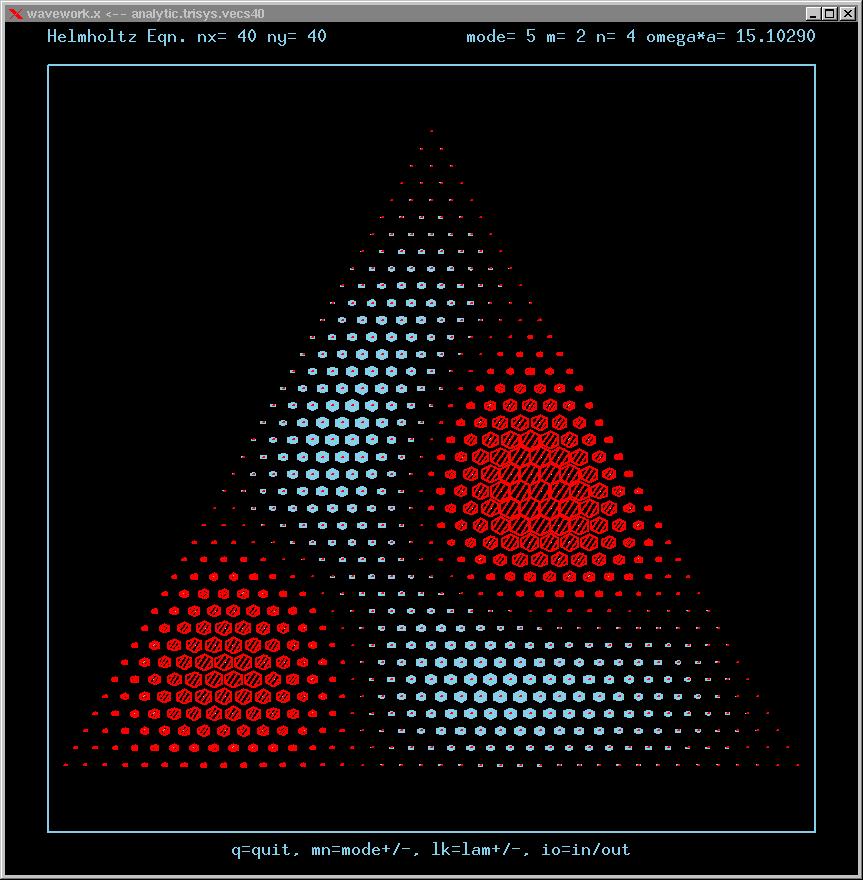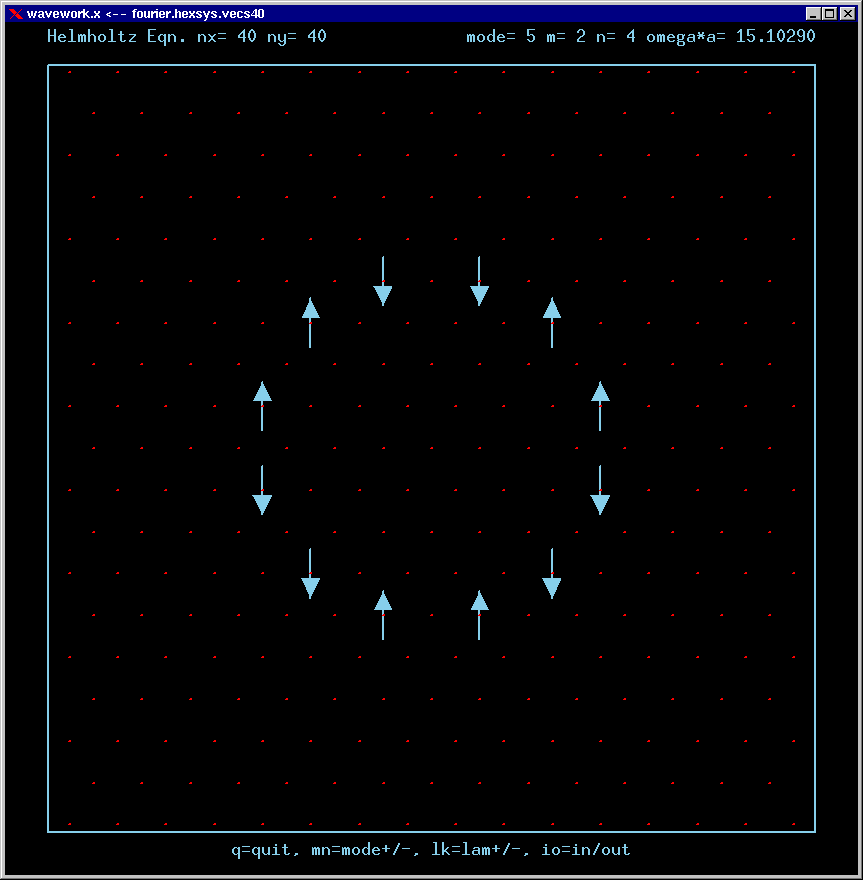Mode 6 (degenerate with 5) and its FT: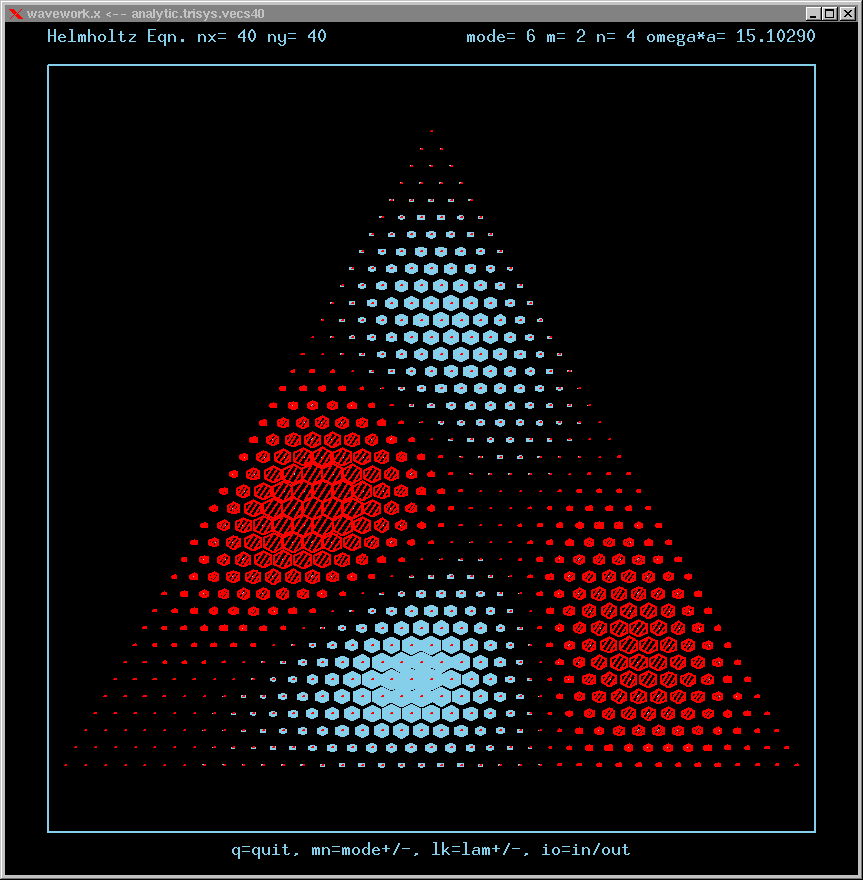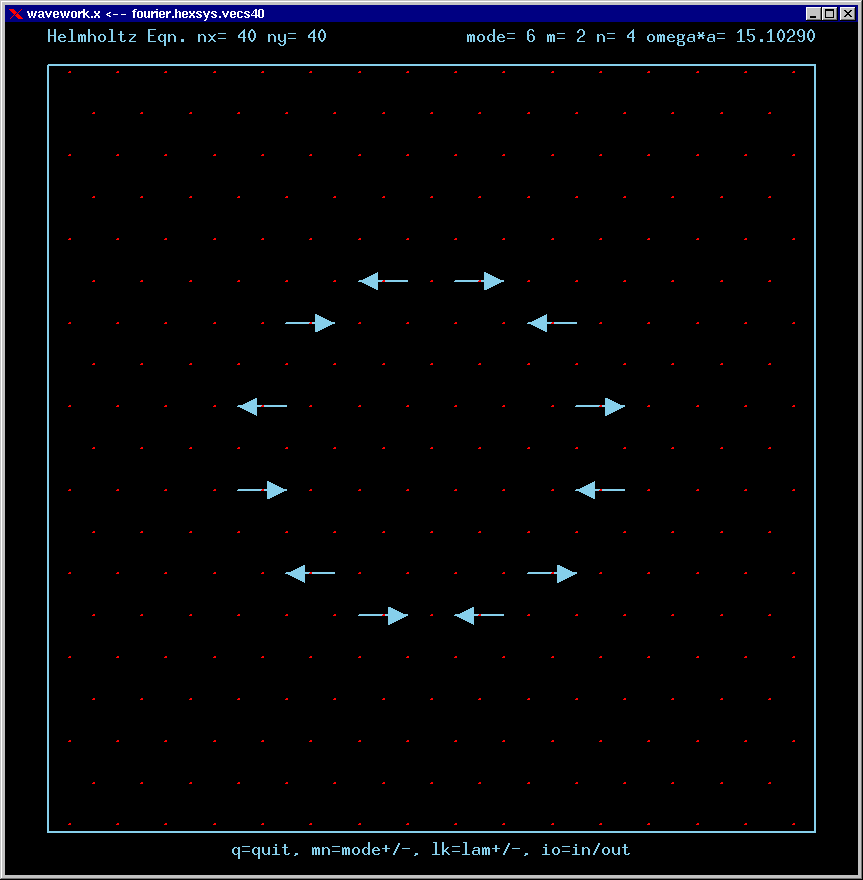Mode 7 (degenerate with 8) and its FT: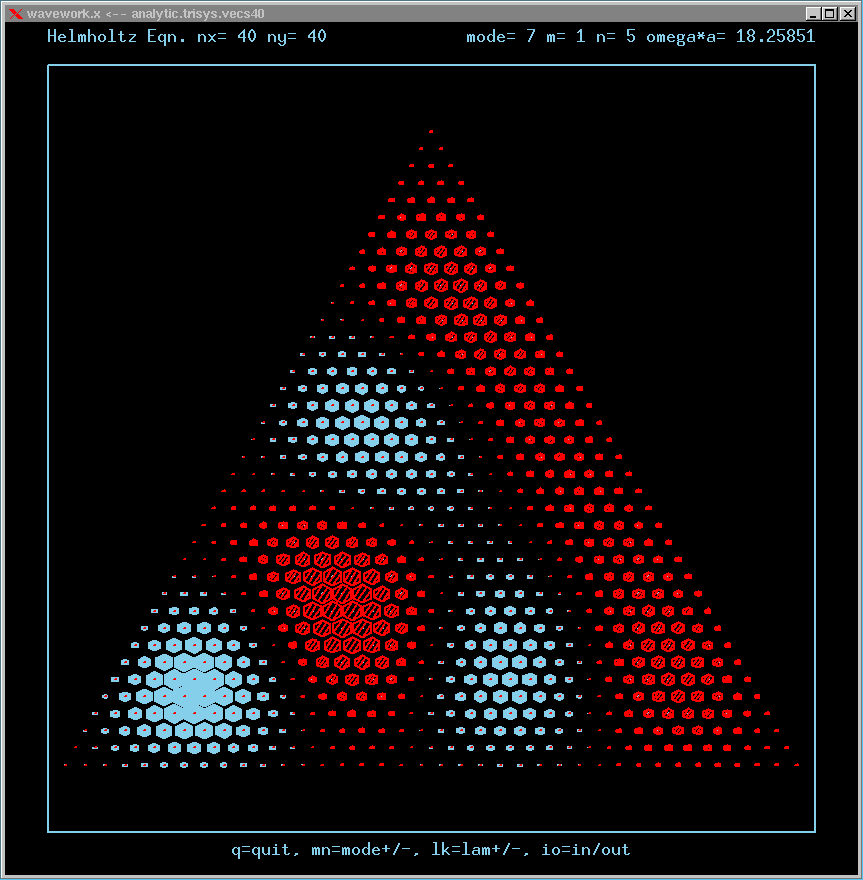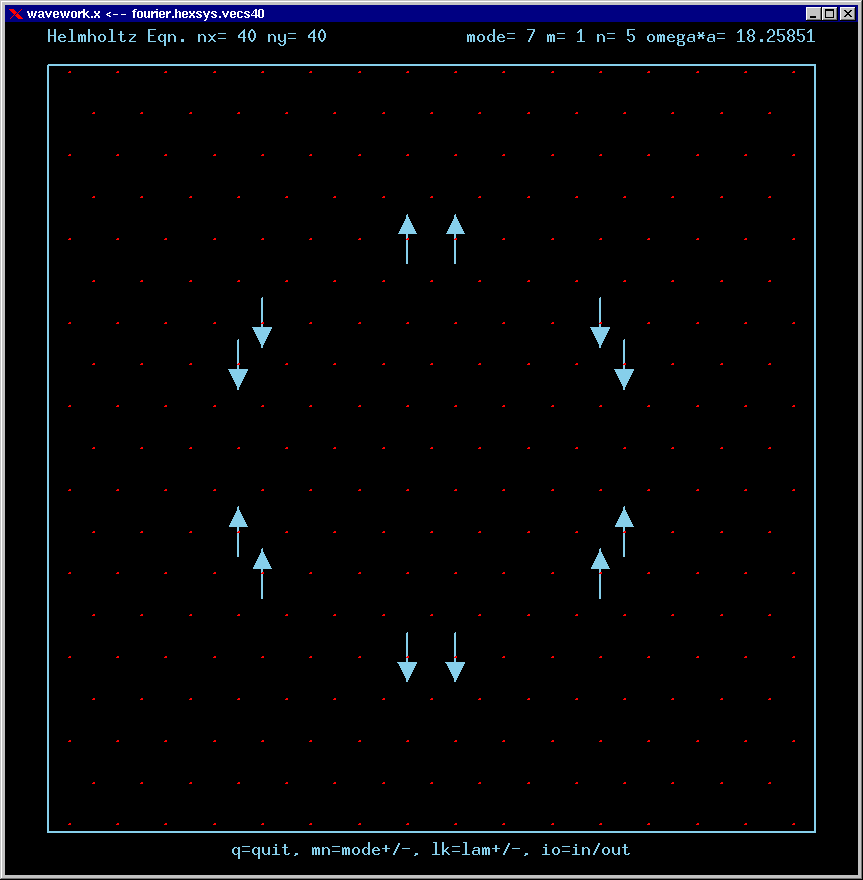Mode 8 (degenerate with 7) and its FT: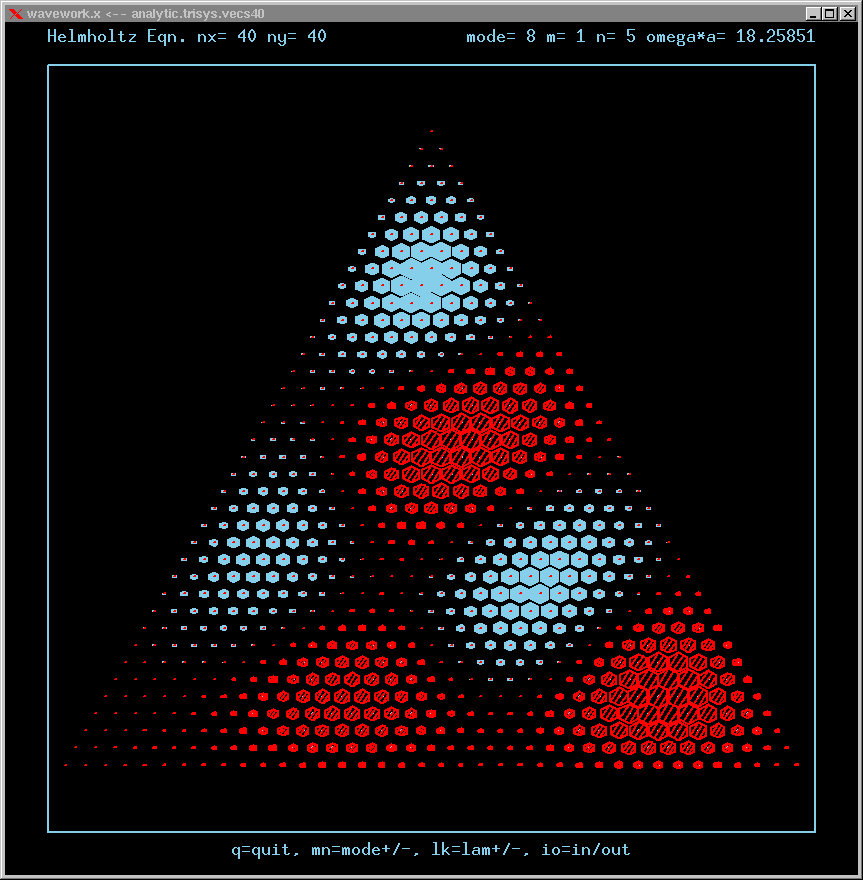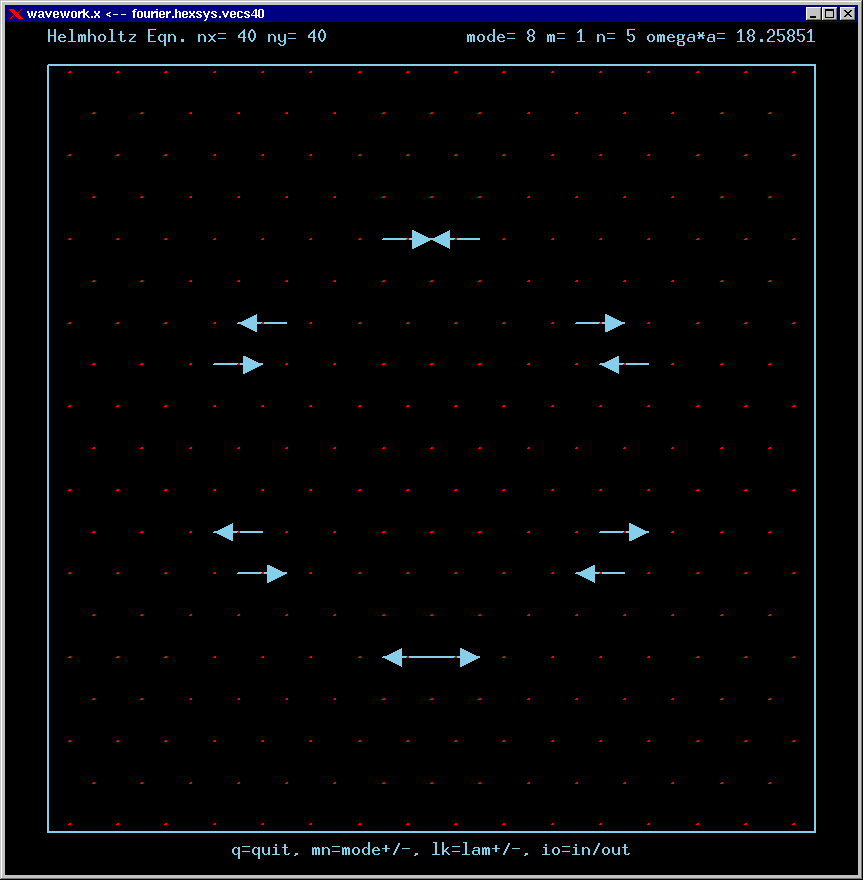Mode 9 (degenerate with 10) and its FT: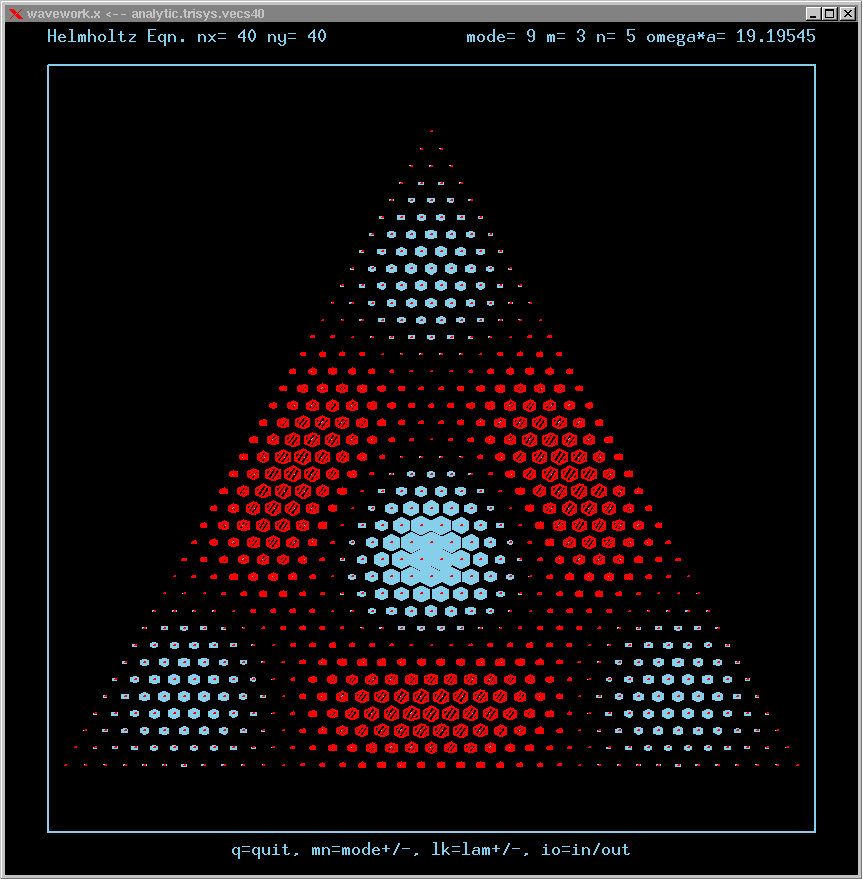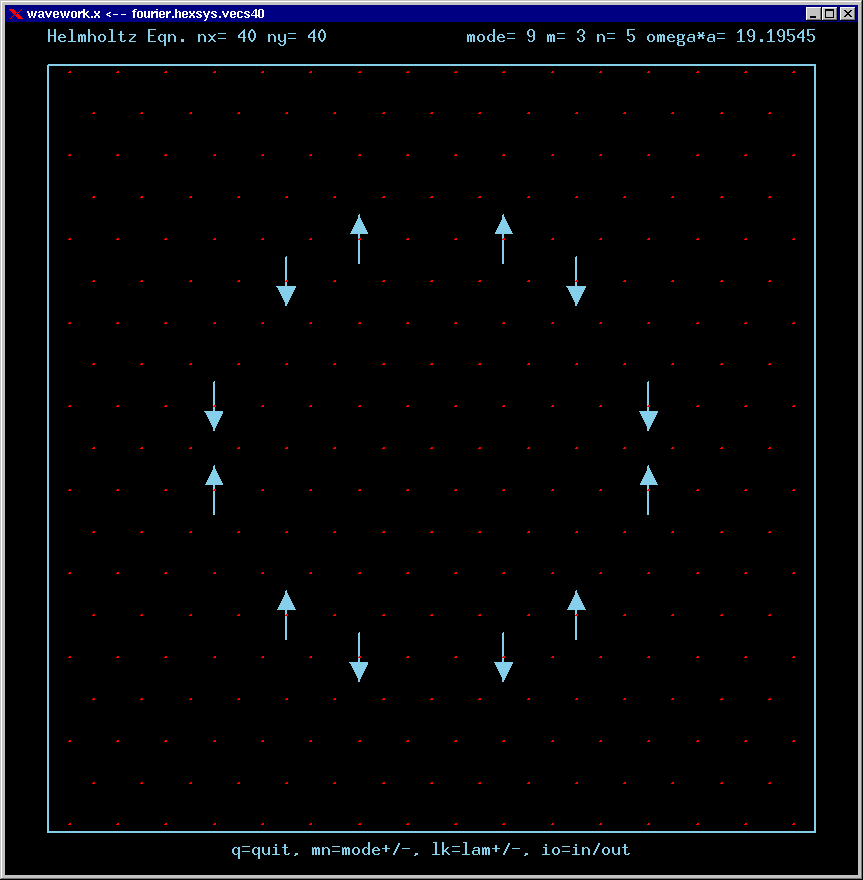Mode 10 (degenerate with 9) and its FT: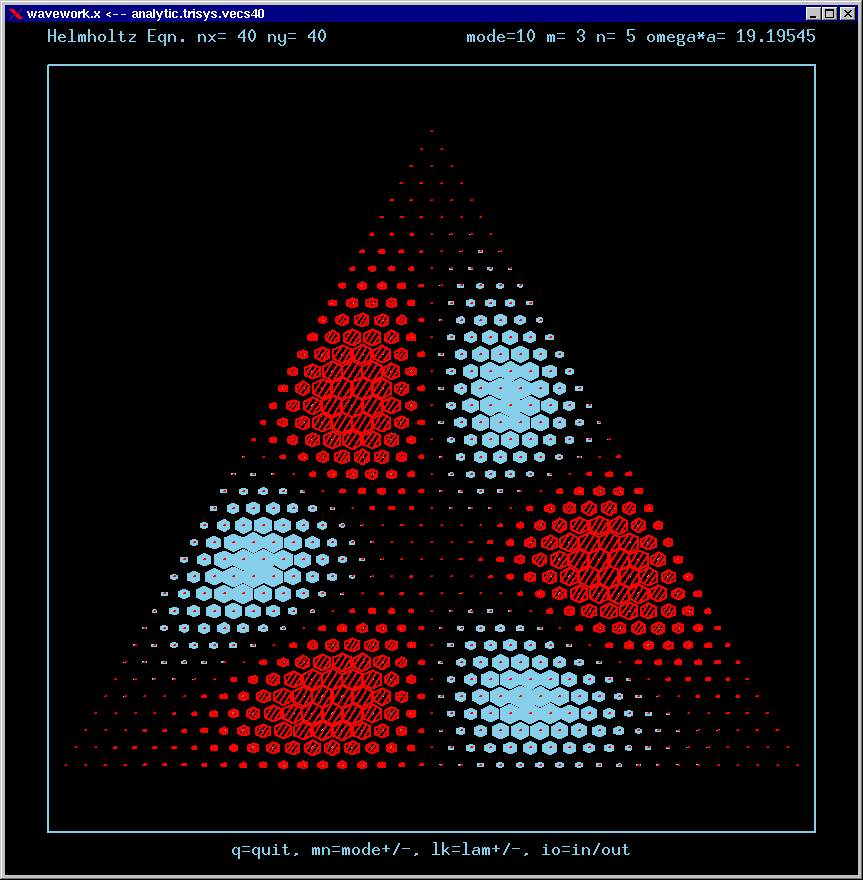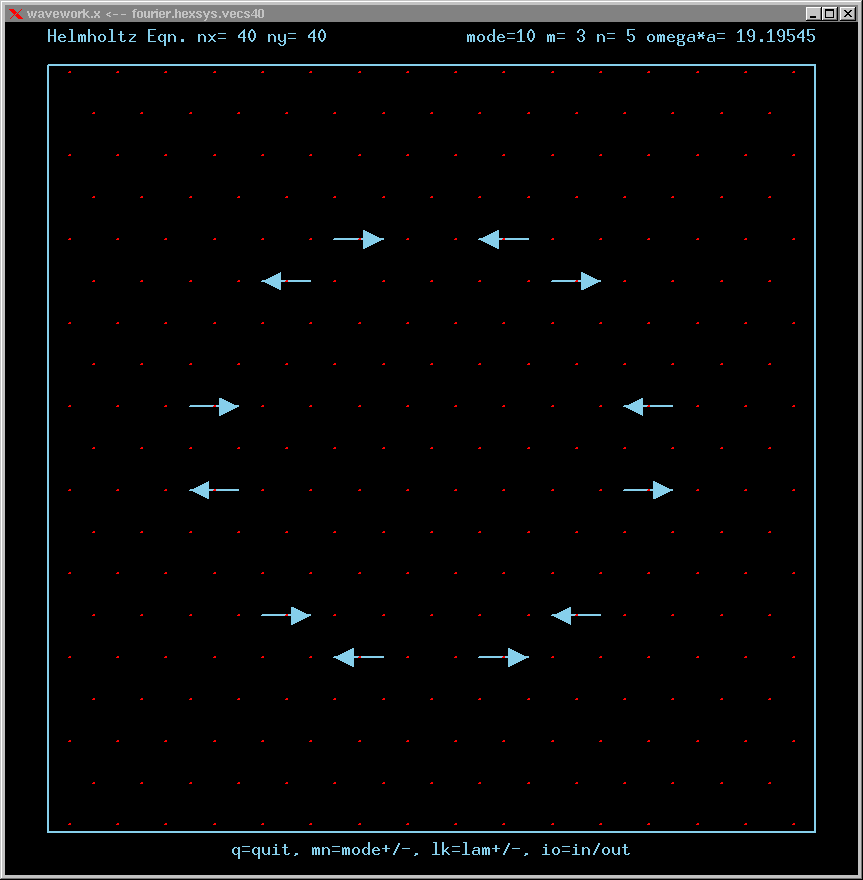Mode 11 (nondegenerate) and its FT: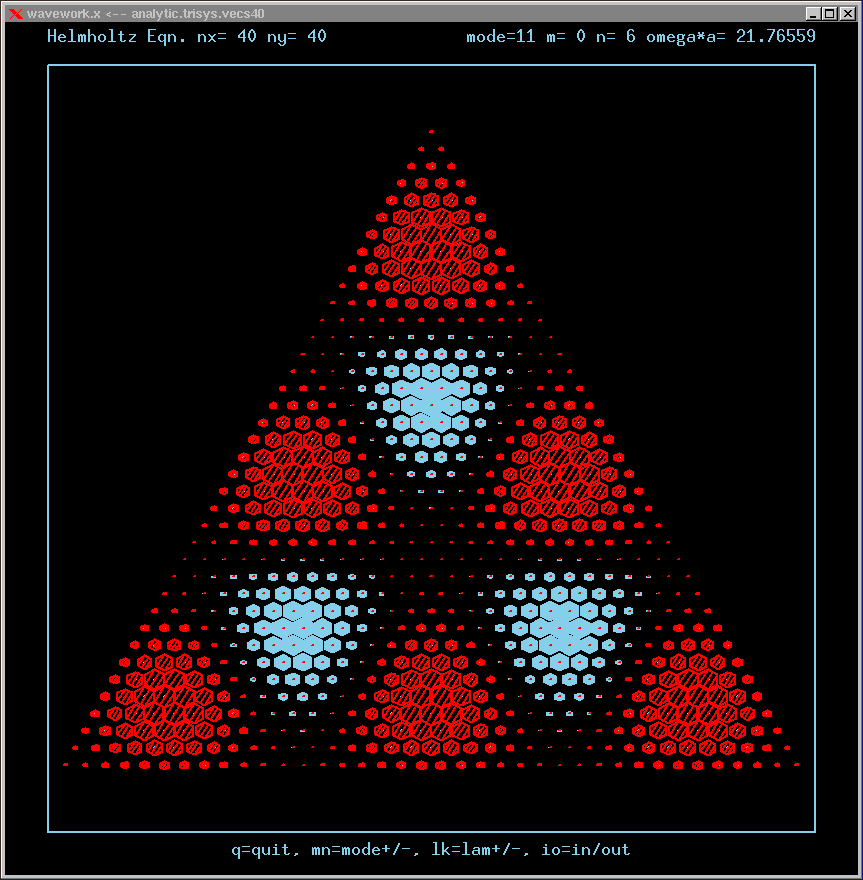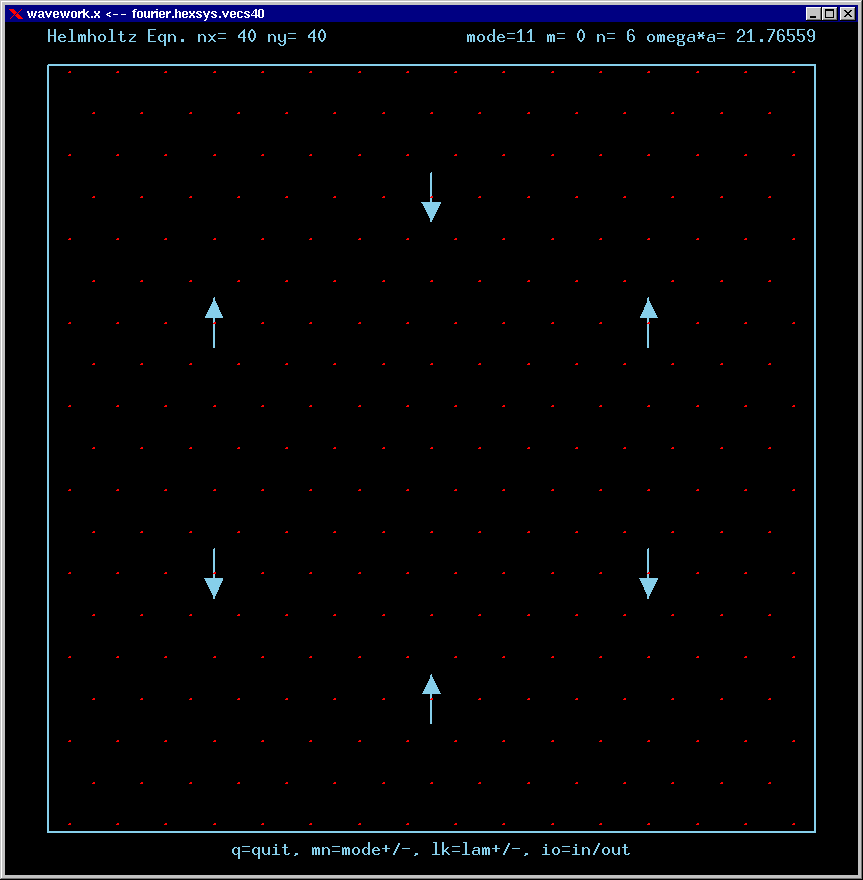Mode 12 (degenerate with 13) and its FT: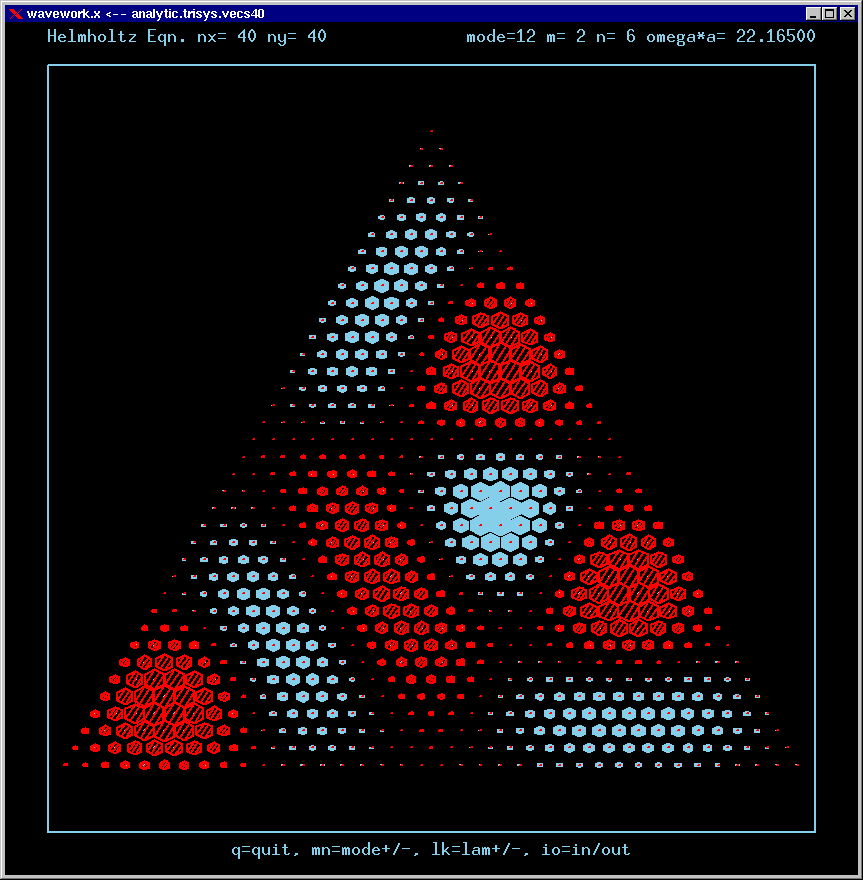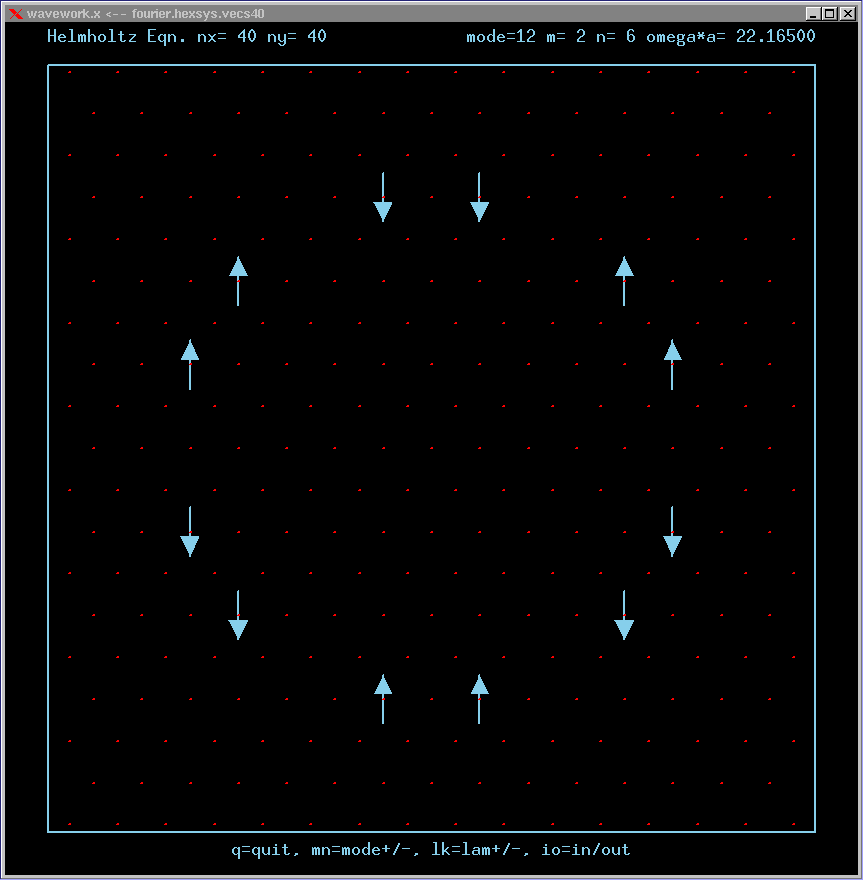Mode 13 (degenerate with 12) and its FT: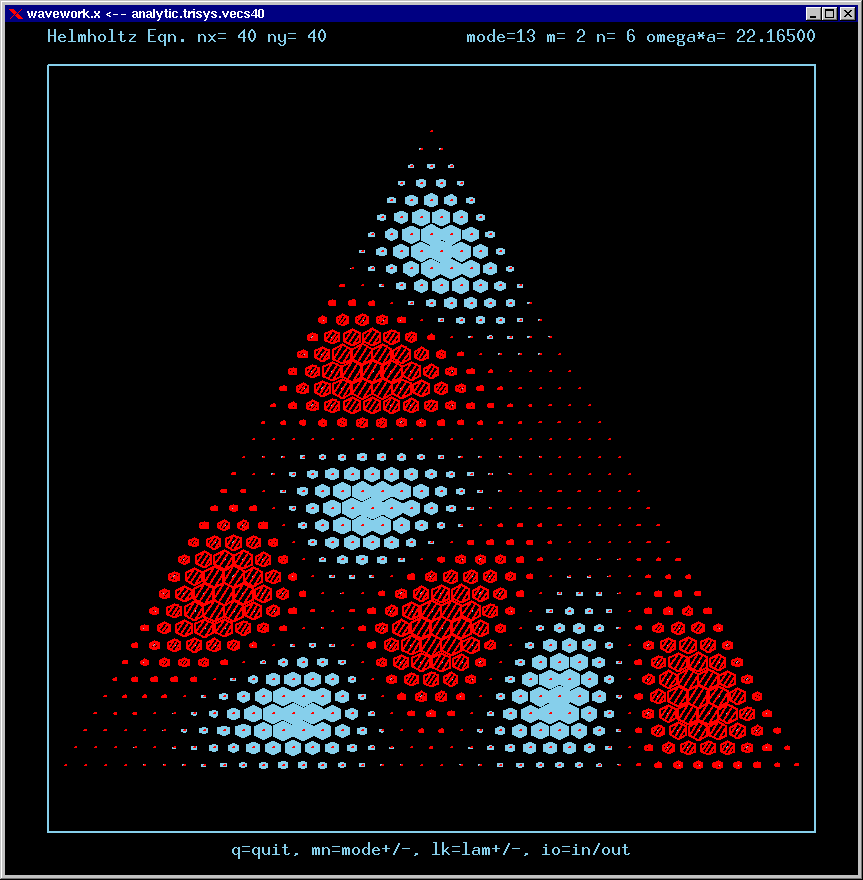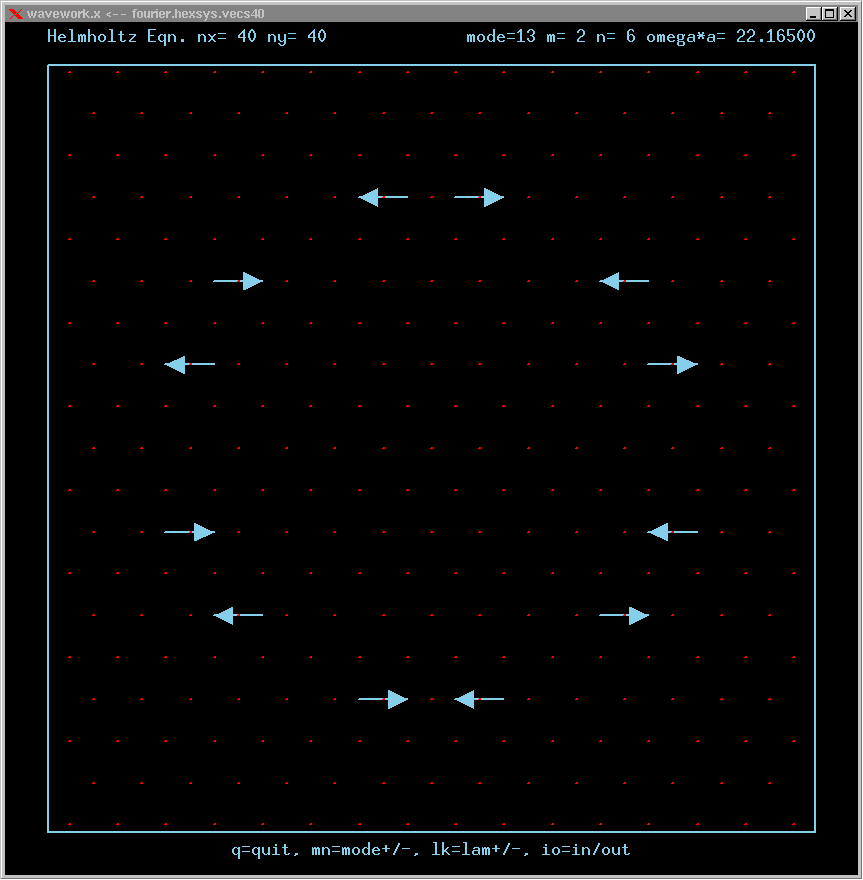Mode 14 (degenerate with 15) and its FT: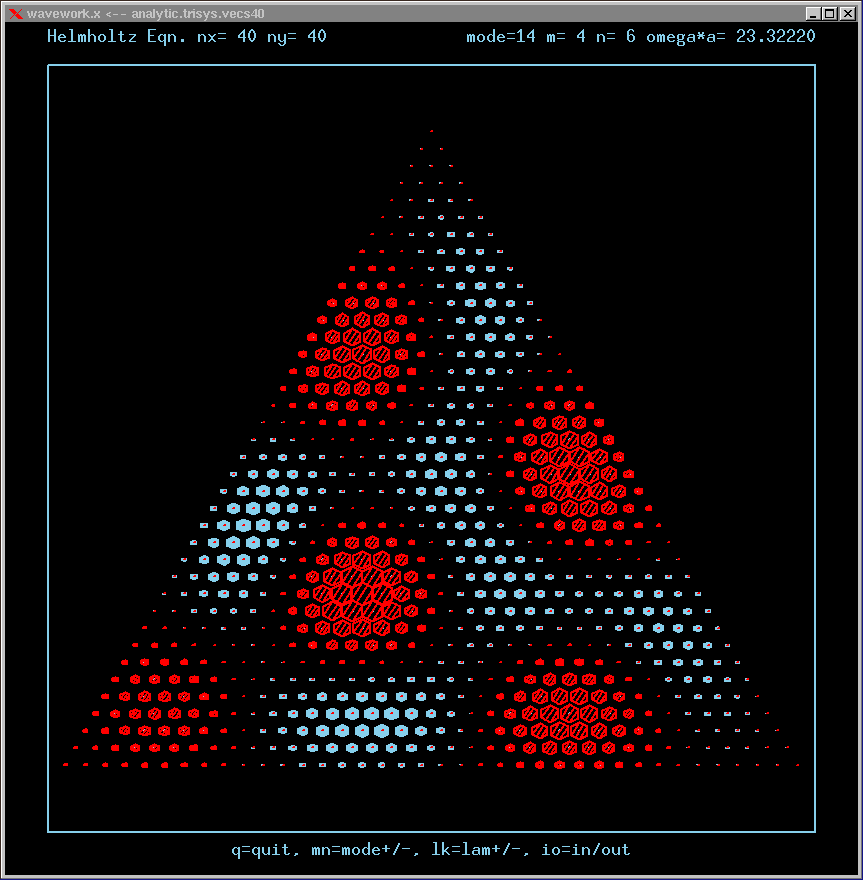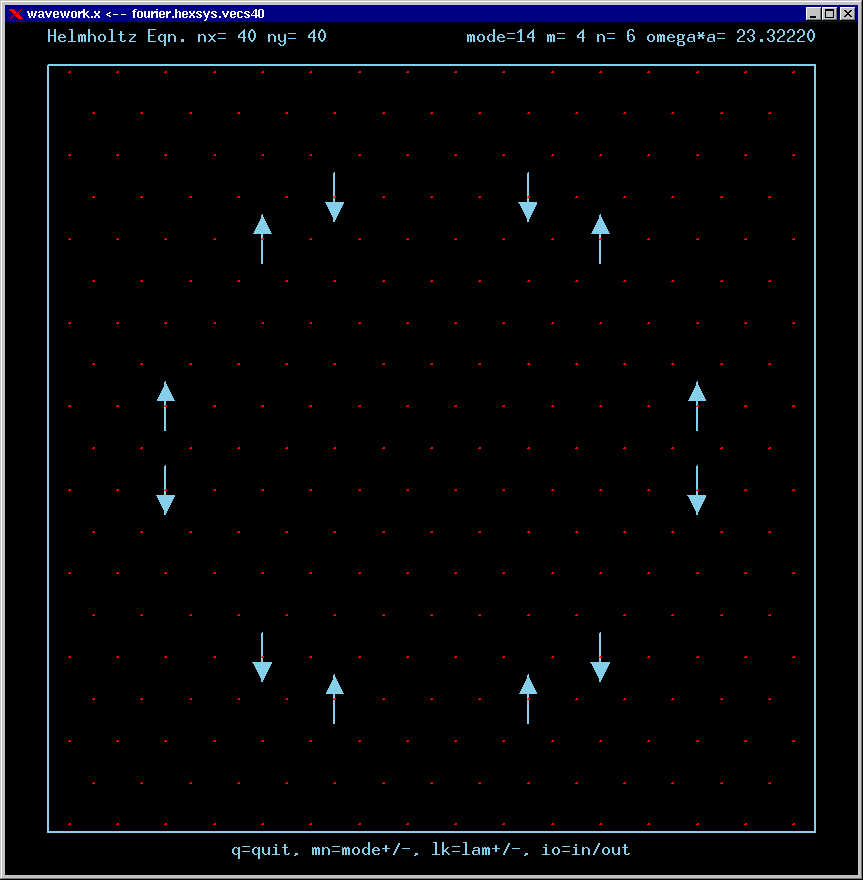Mode 15 (degenerate with 14) and its FT: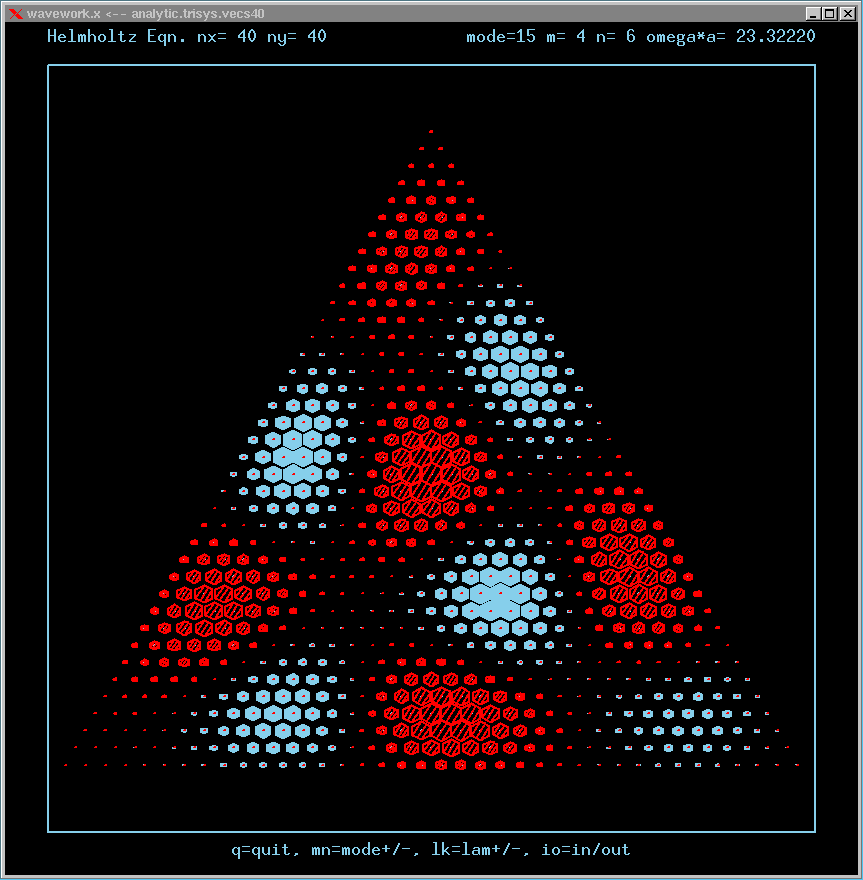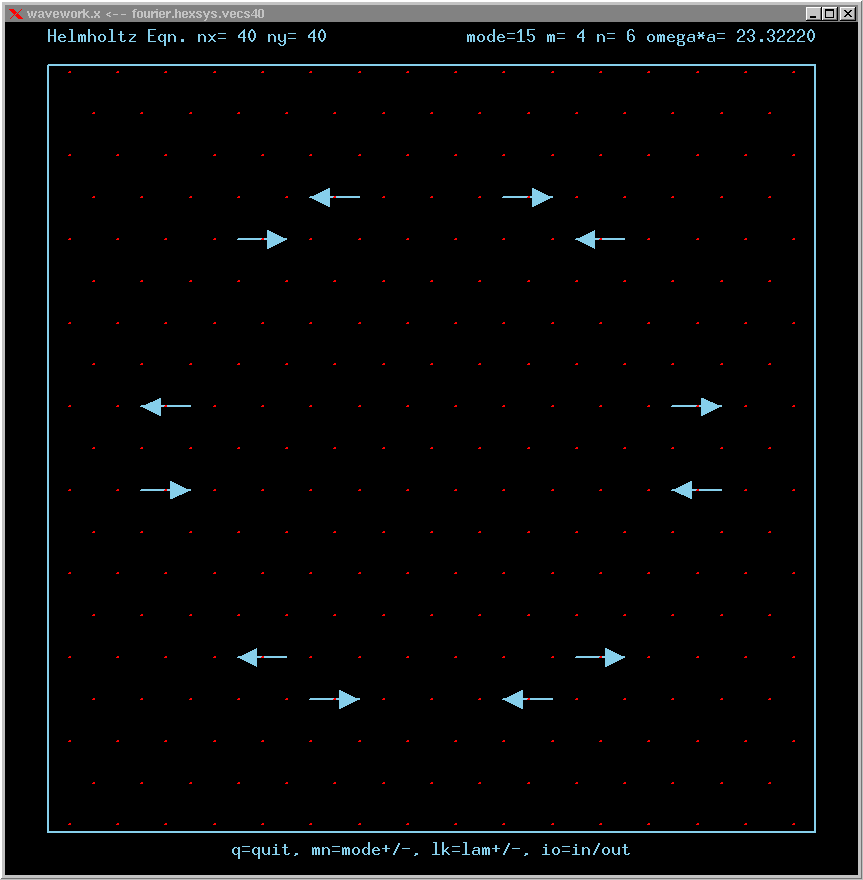Mode 16 (degenerate with 17) and its FT: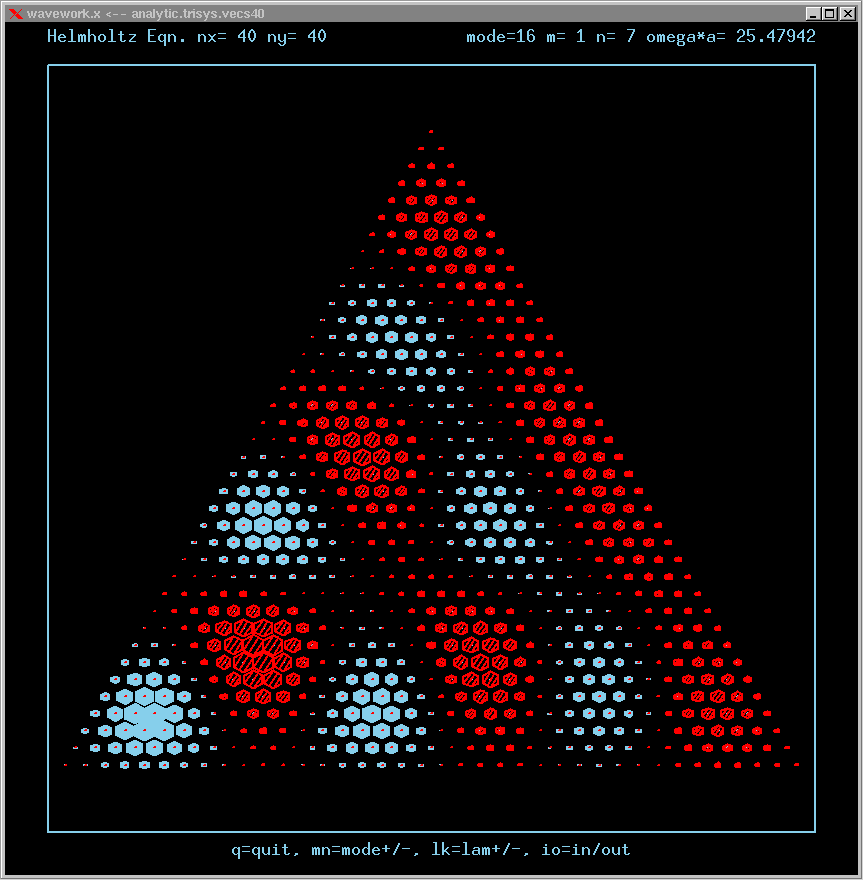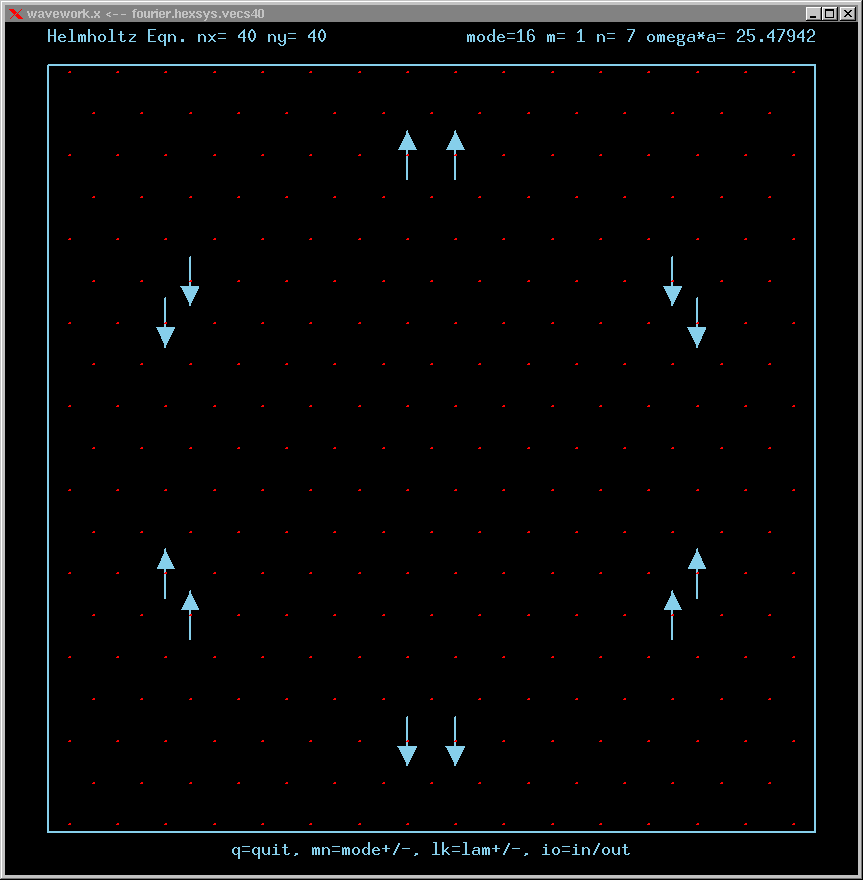Mode 17 (degenerate with 16) and its FT: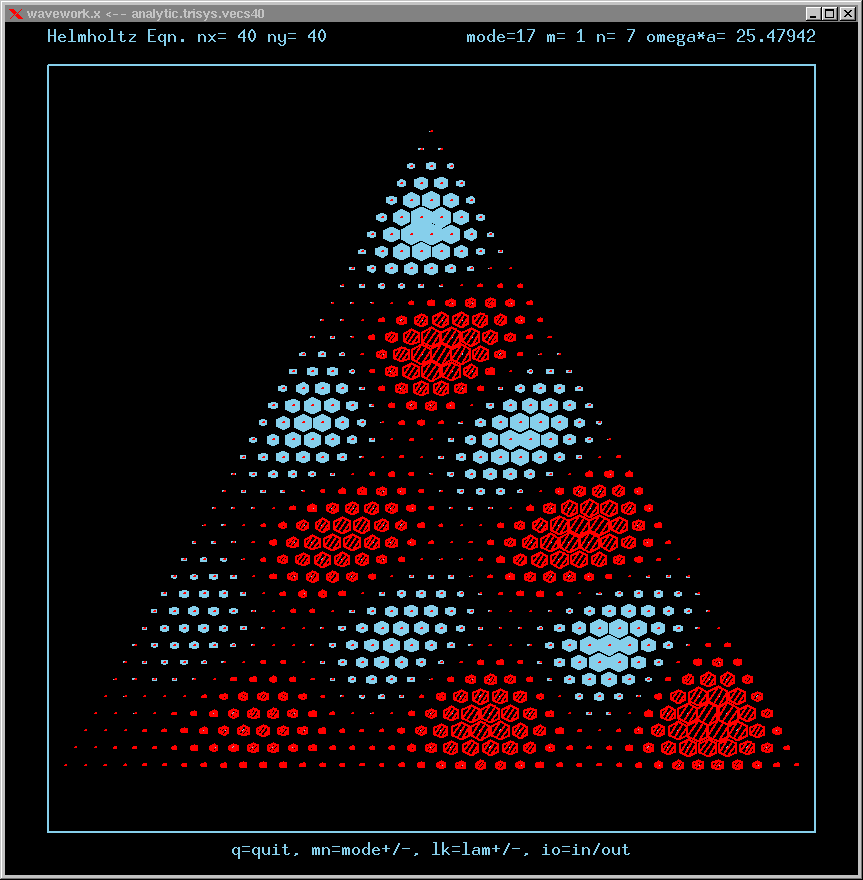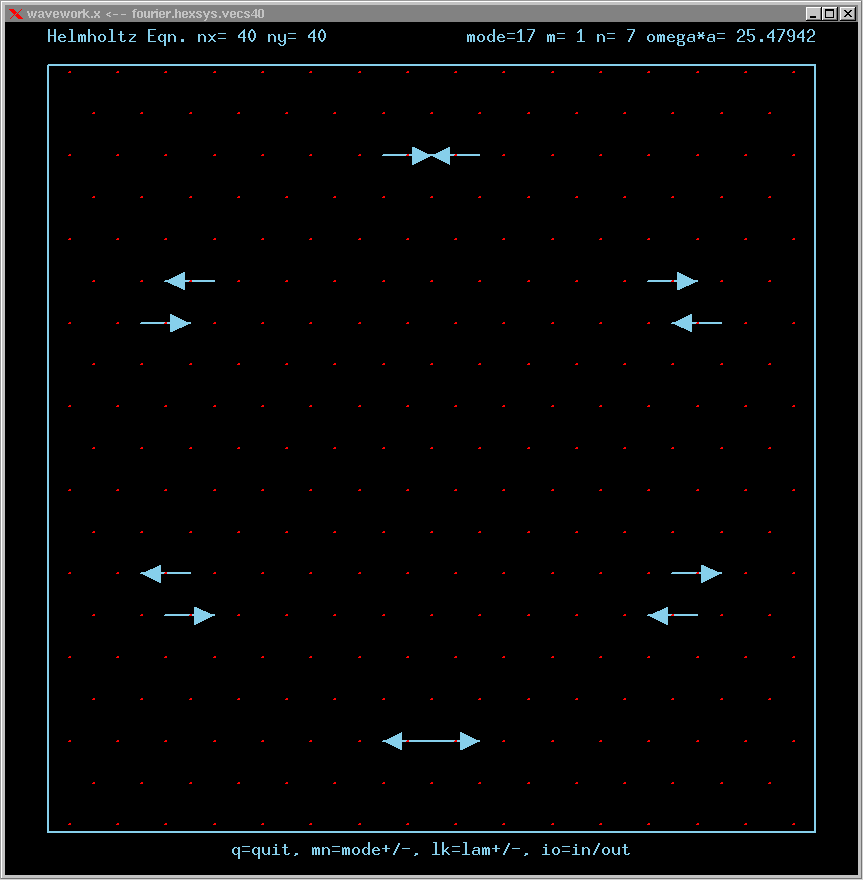Mode 18 (degenerate with 19) and its FT: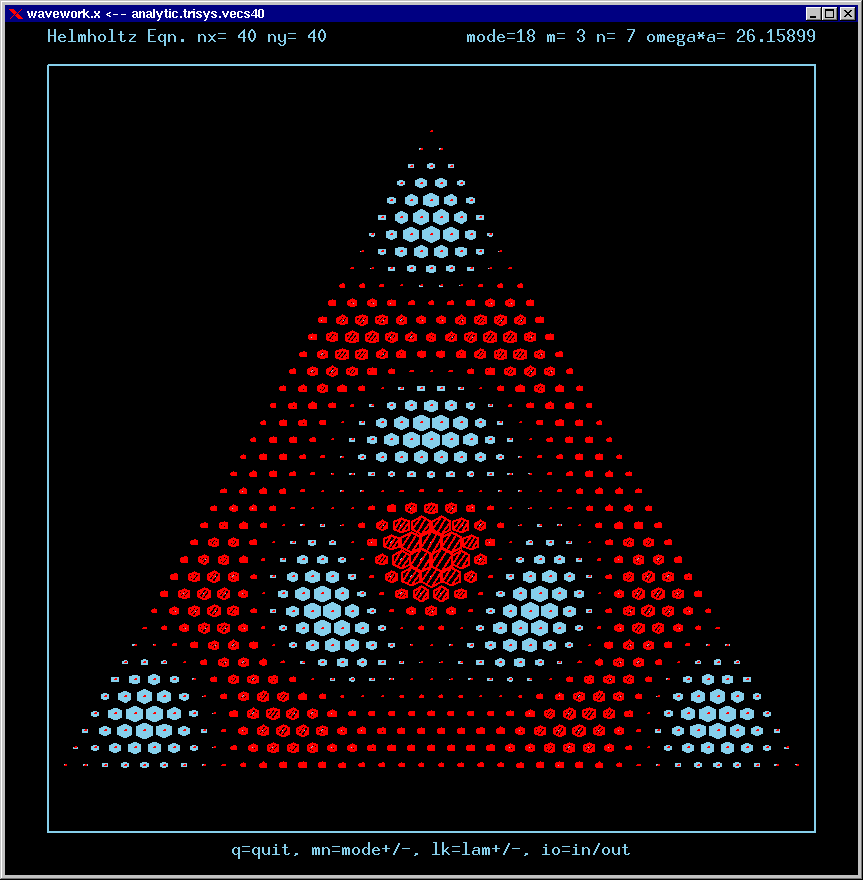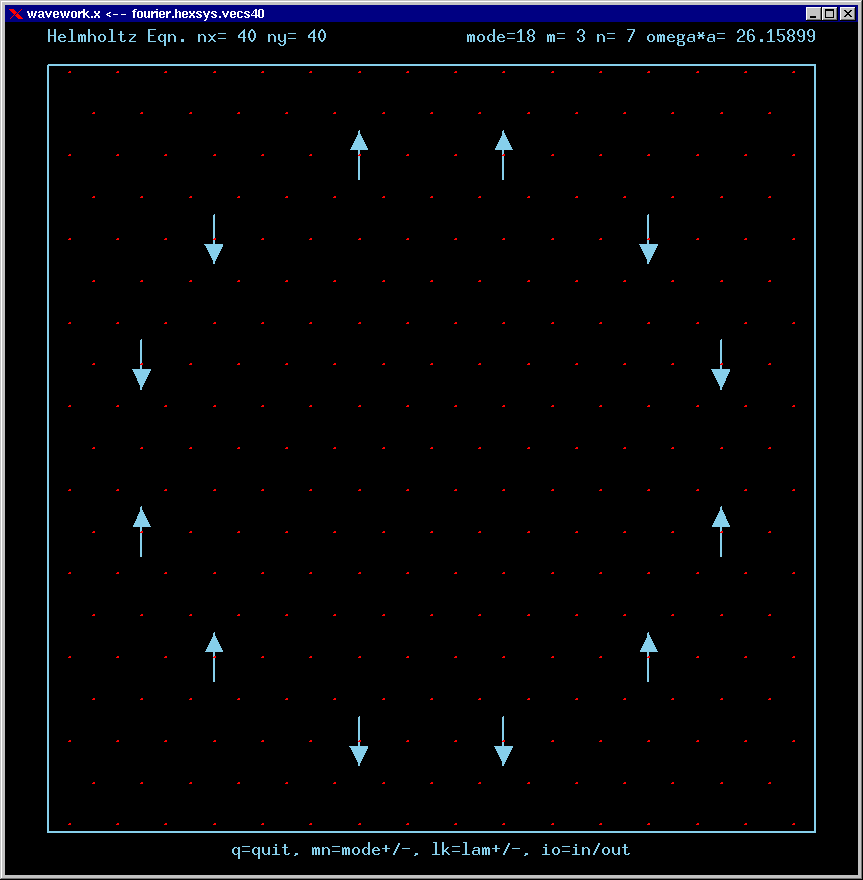Mode 19 (degenerate with 18) and its FT: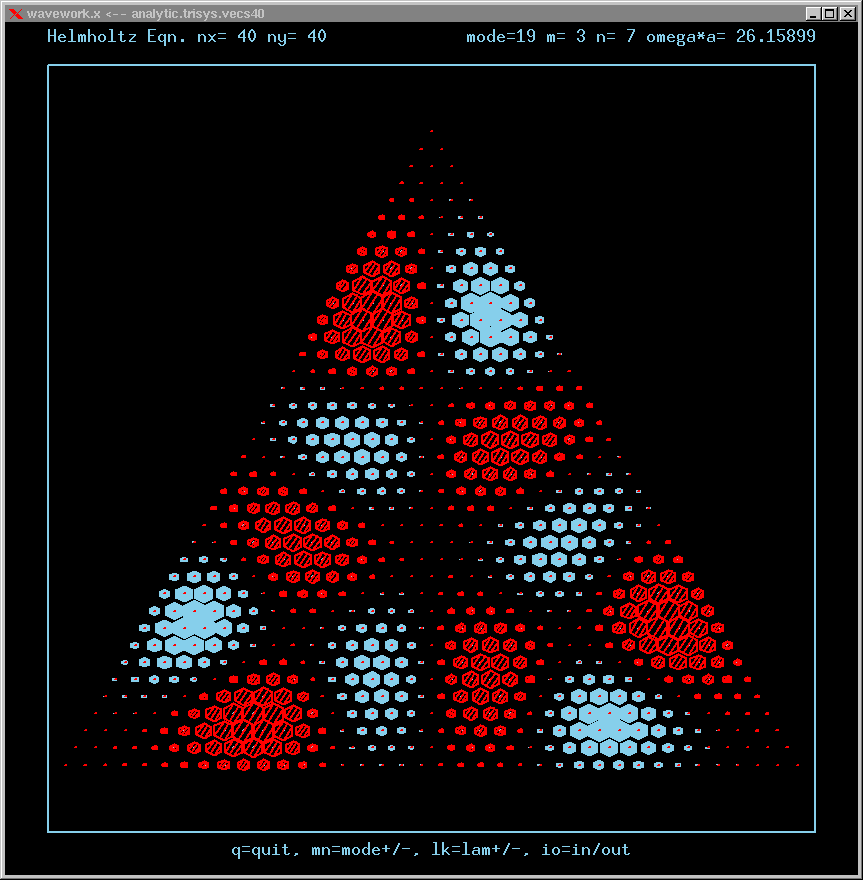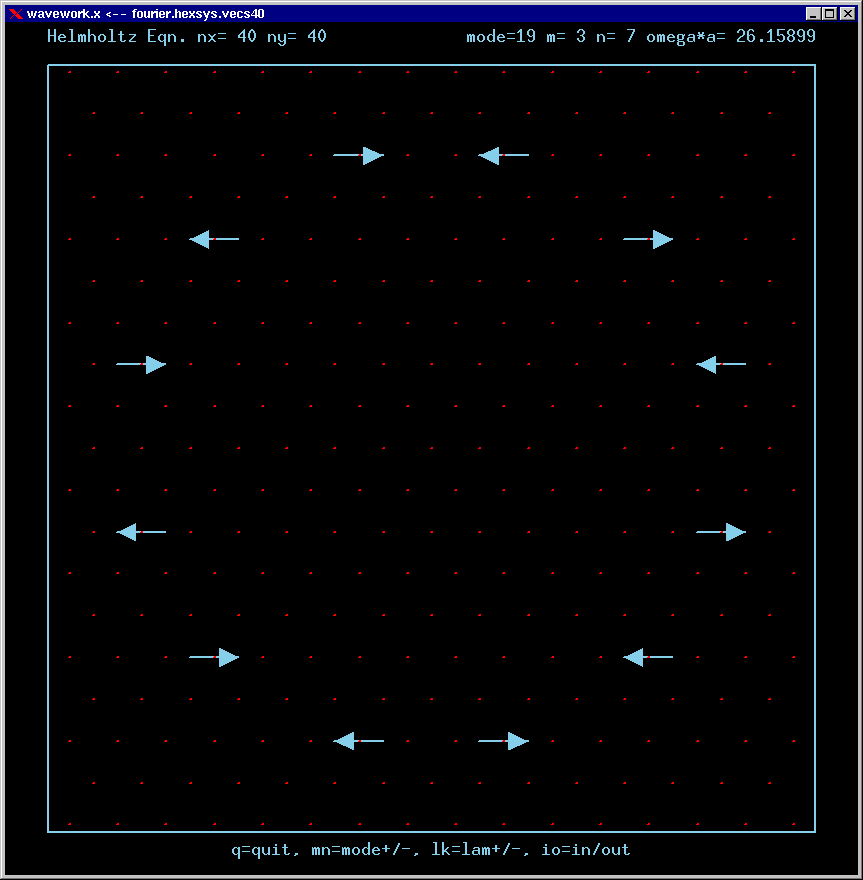Mode 20 (degenerate with 21) and its FT: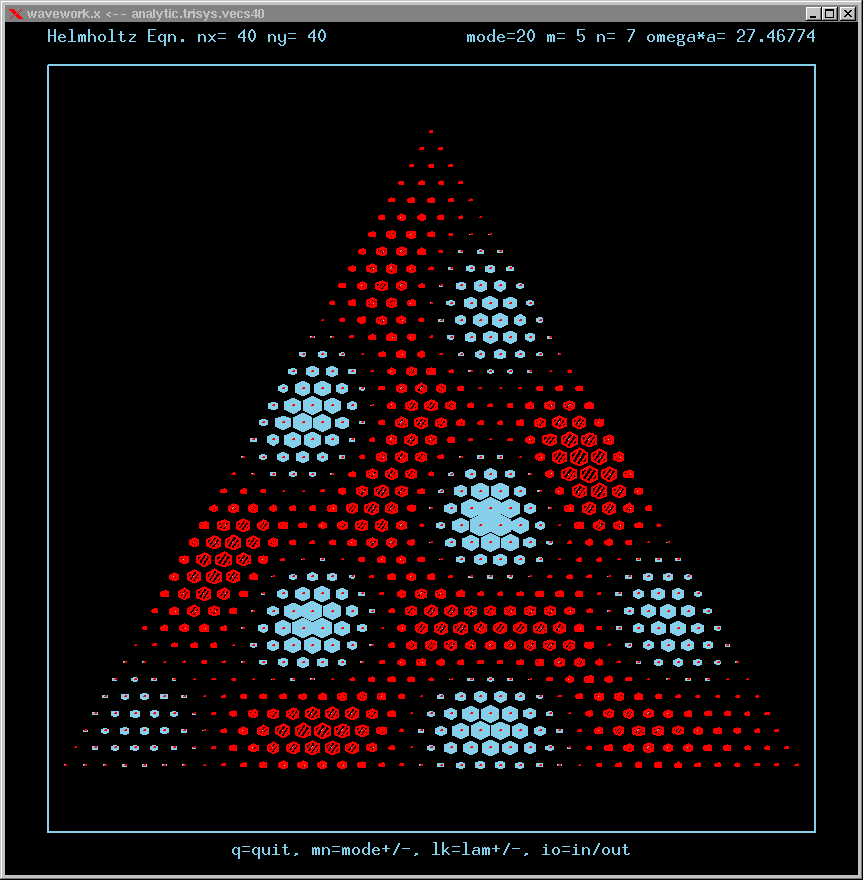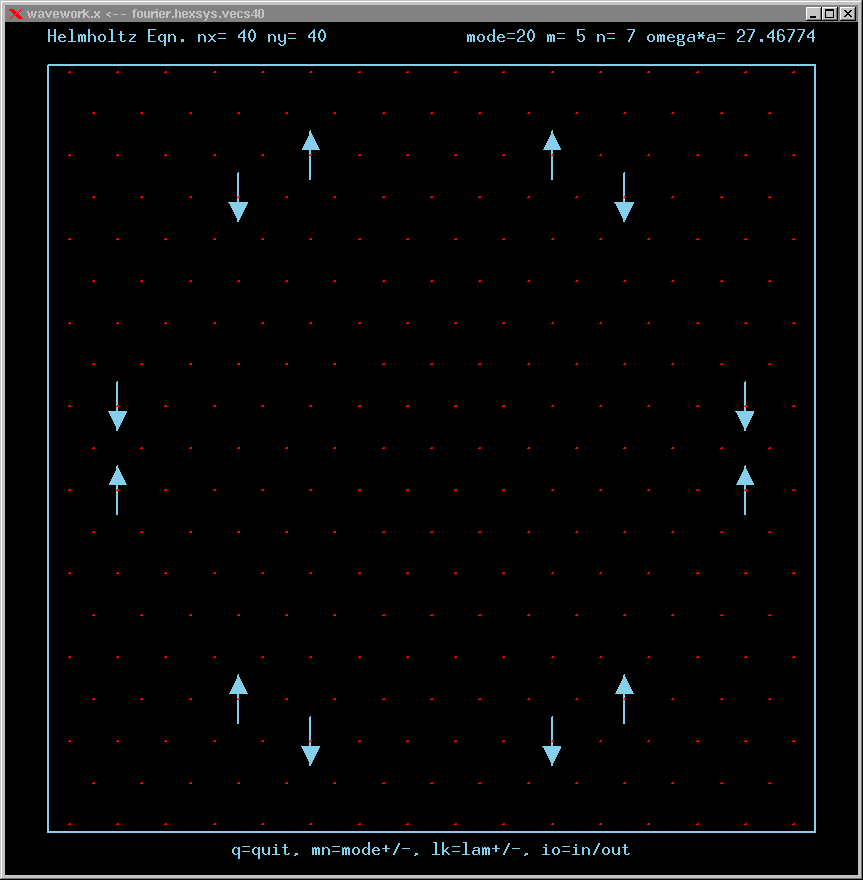Mode 21 (degenerate with 20) and its FT: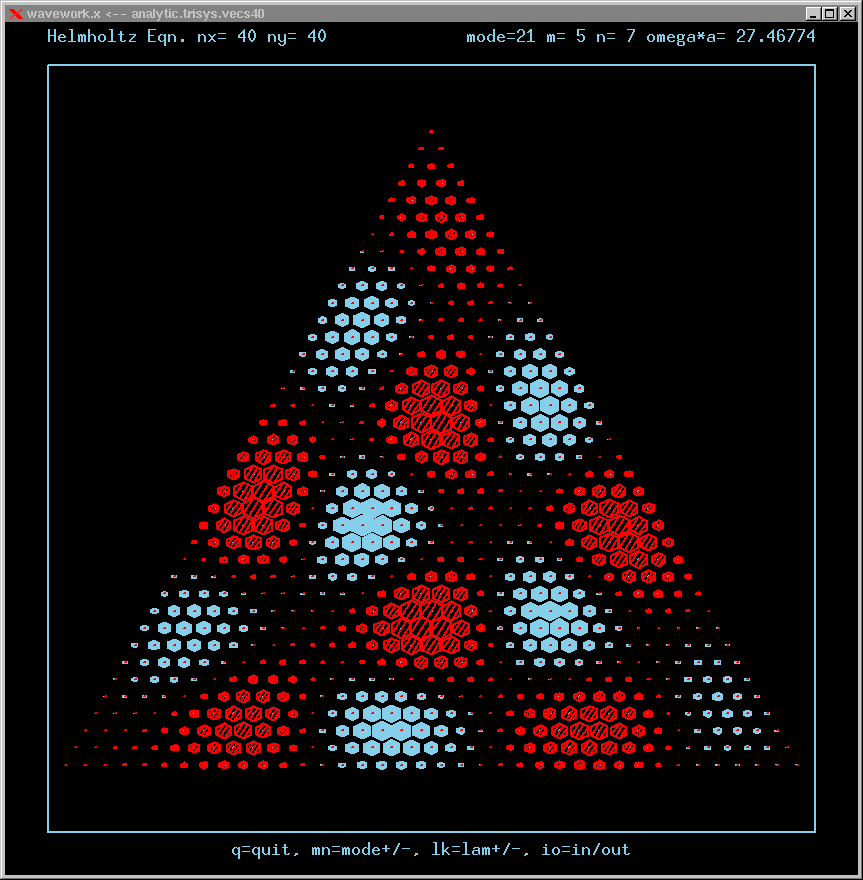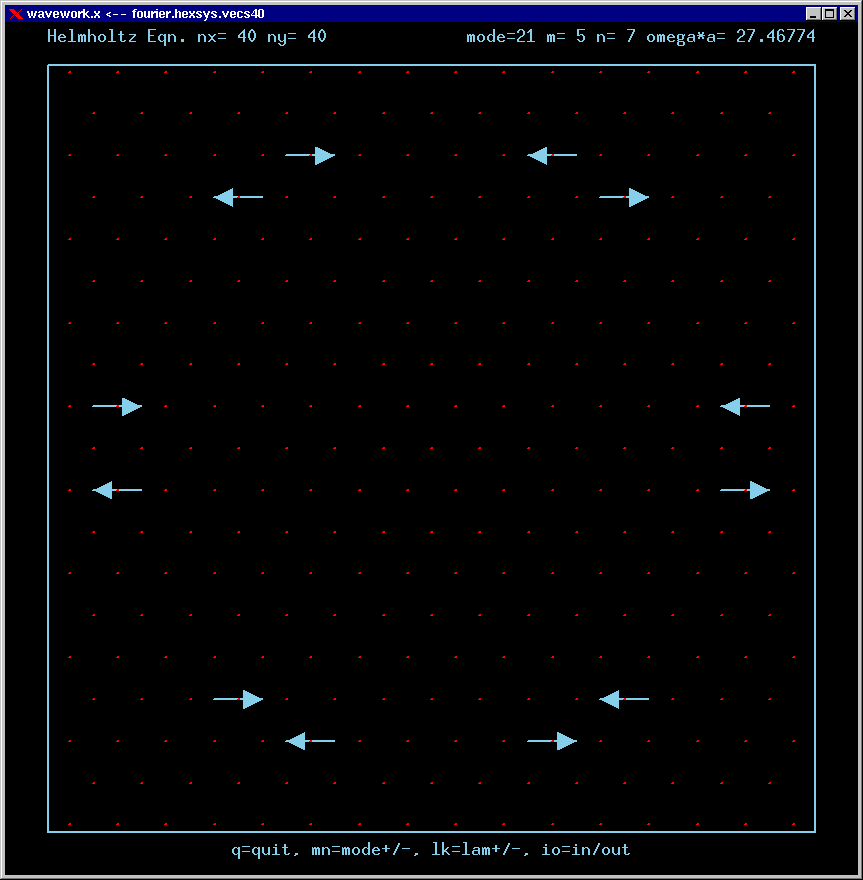Mode 22 (nondegenerate) and its FT: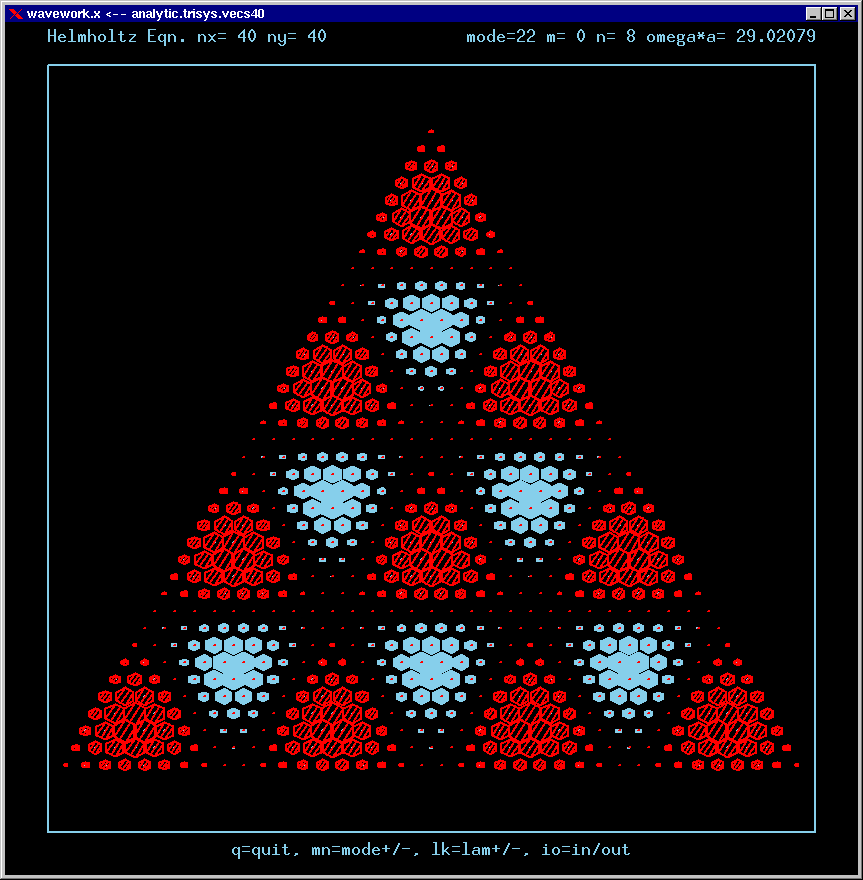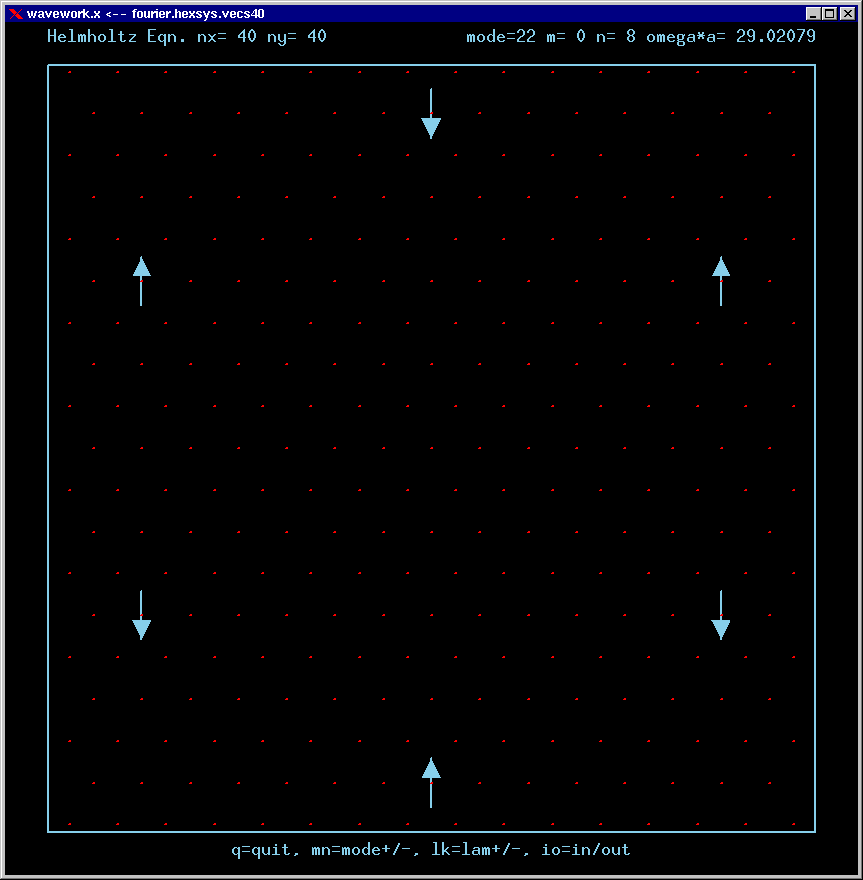Mode 23 (degenerate with 24) and its FT: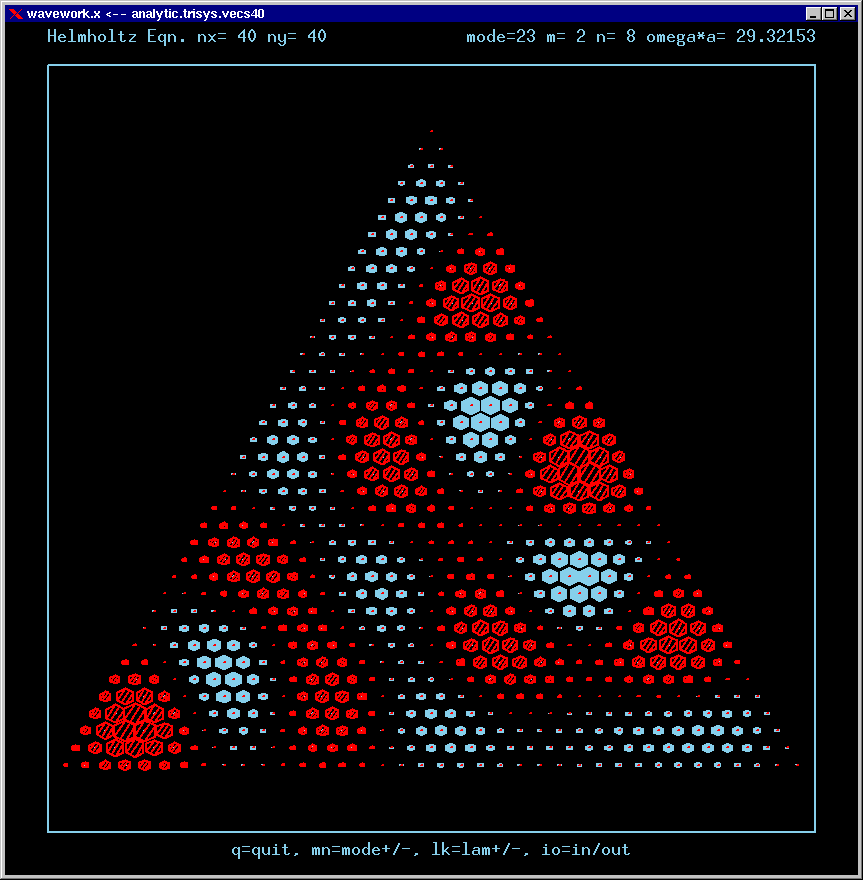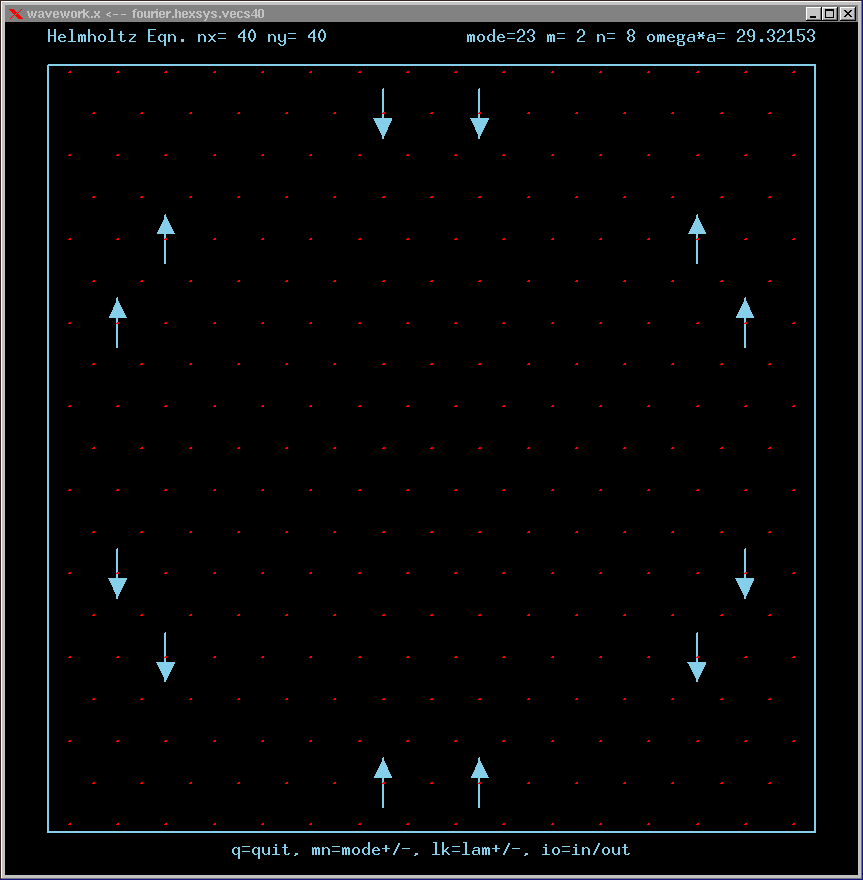Mode 24 (degenerate with 23) and its FT: